# CBSE Class 10 Maths Question Paper 2020

CBSE Class 10 Maths exam was conducted on 12th March 2020. This year for the first time CBSE has conducted the two different Maths papers i.e “Mathematics Standard” and “Mathematics Basics”. Students who want to pursue their career ahead in mathematics have opted for the Math Standard and those who don’t want to move ahead with mathematics have chosen for Maths Basics. Here we have provided the CBSE Class 10 Maths Question Paper 2020 for both Standard Maths. We will be soon updating the Basic Maths Question paper as well.

## CBSE Class 10 Maths Question Paper 2020 – All Sets

Click on the link below to access the CBSE Class 10 Maths Question Papers 2020 for all types of sets.

CBSE Class 10 Maths Standard Question Paper 2020 Set 1 (30/5/1)

CBSE Class 10 Maths Standard Question Paper 2020 Set 2 (30/5/2)

CBSE Class 10 Maths Standard Question Paper 2020 Set 3 (30/5/3)

## CBSE Class 10 Mathematics Question Paper 2020 Set 1 (30/5/1)

Students can have a look at the CBSE Class 10 Maths Question Paper 2020 Set 1 below: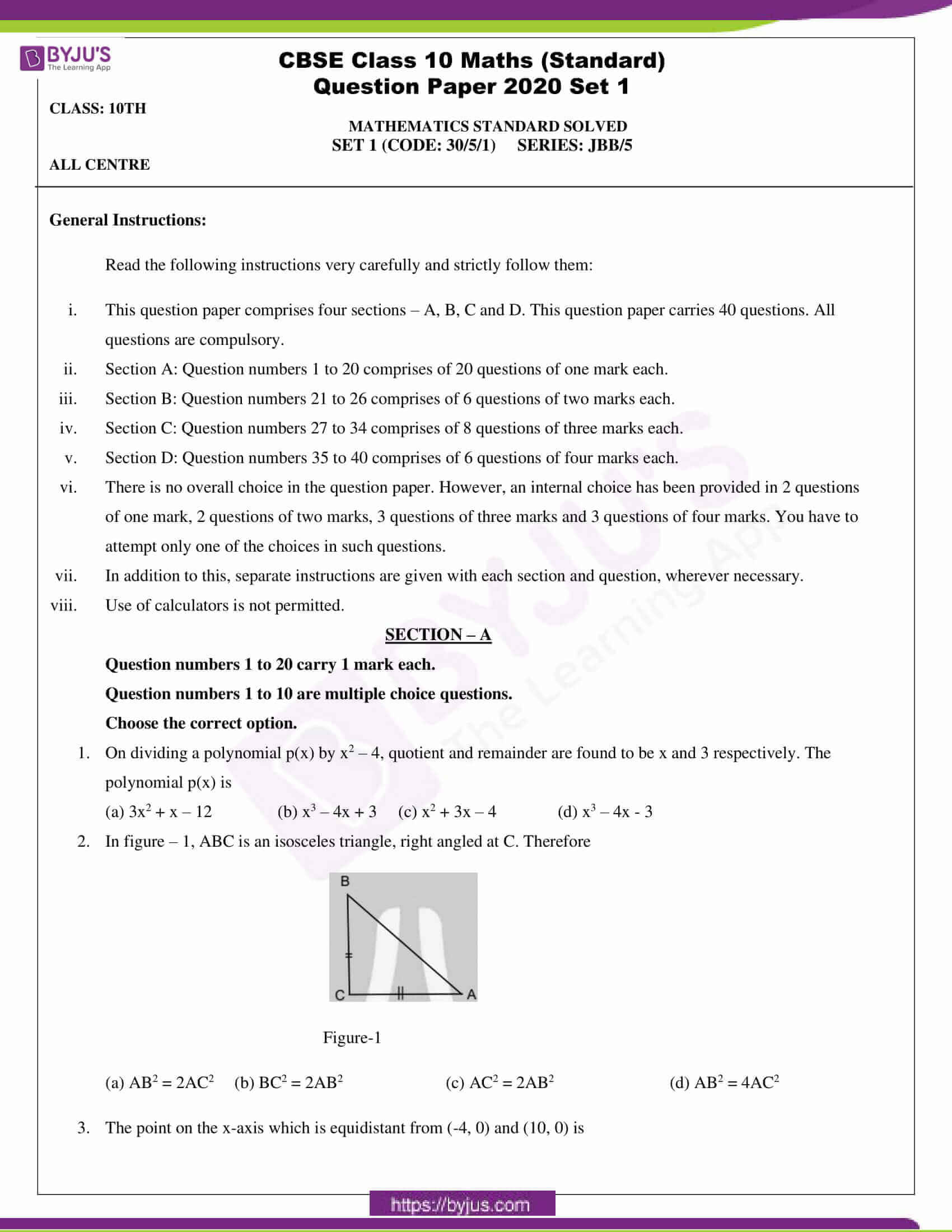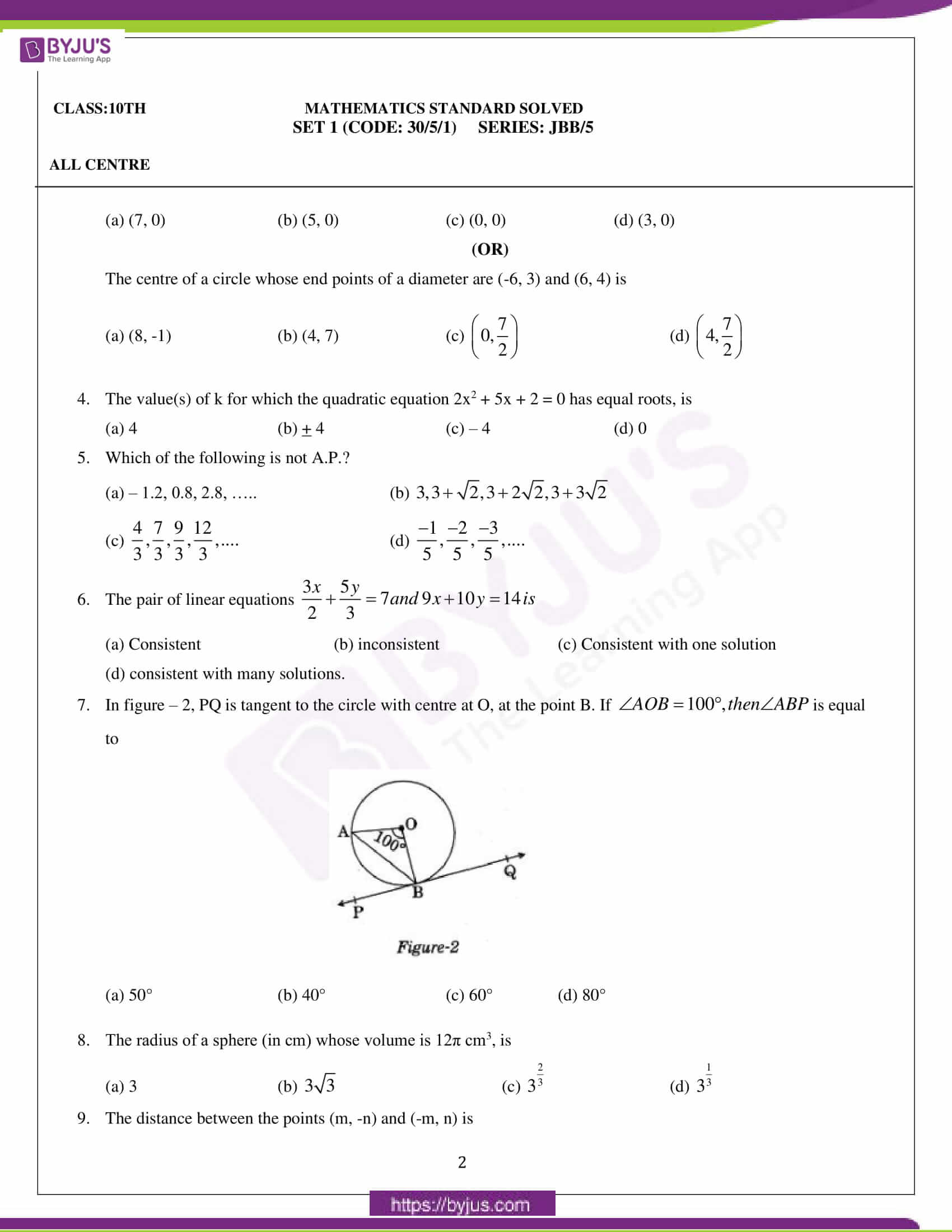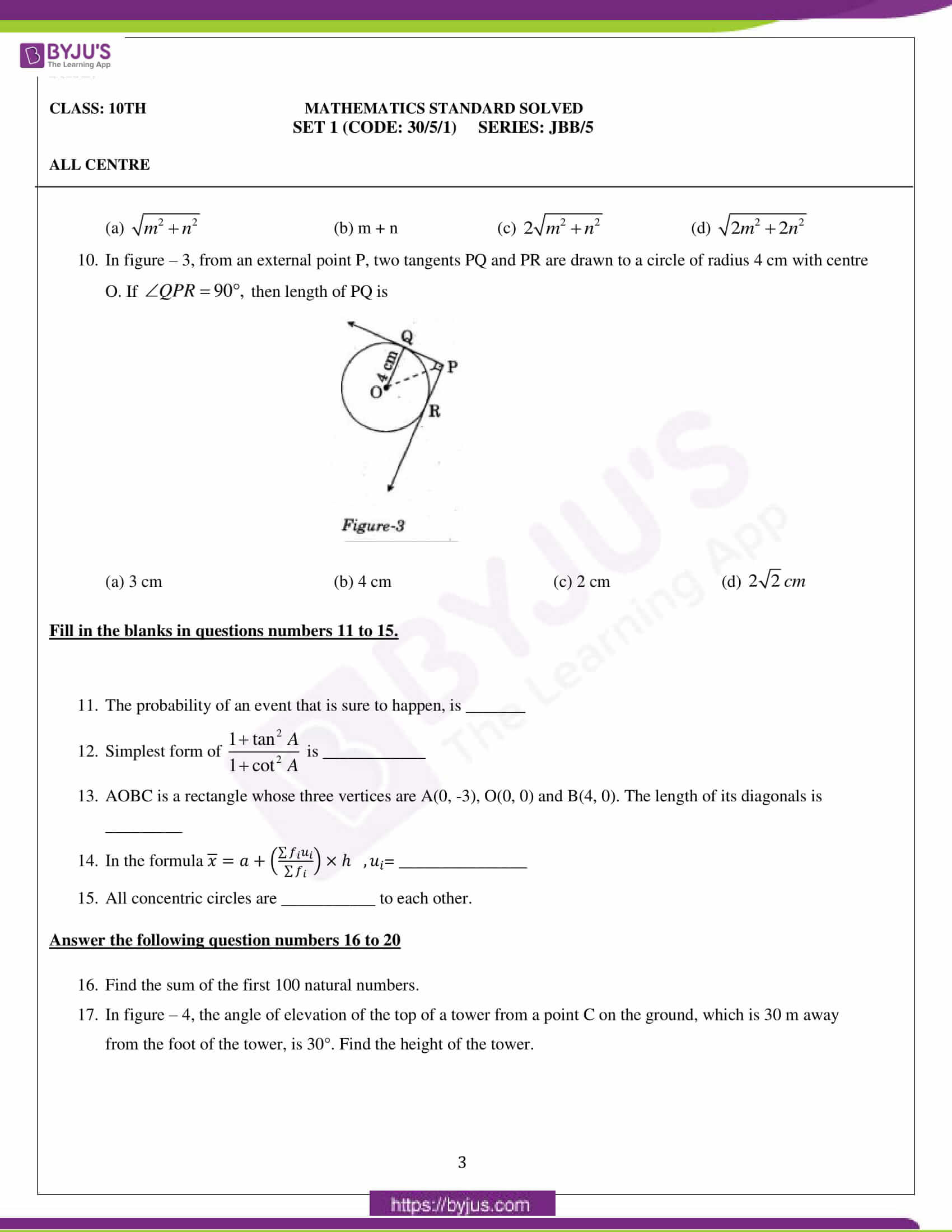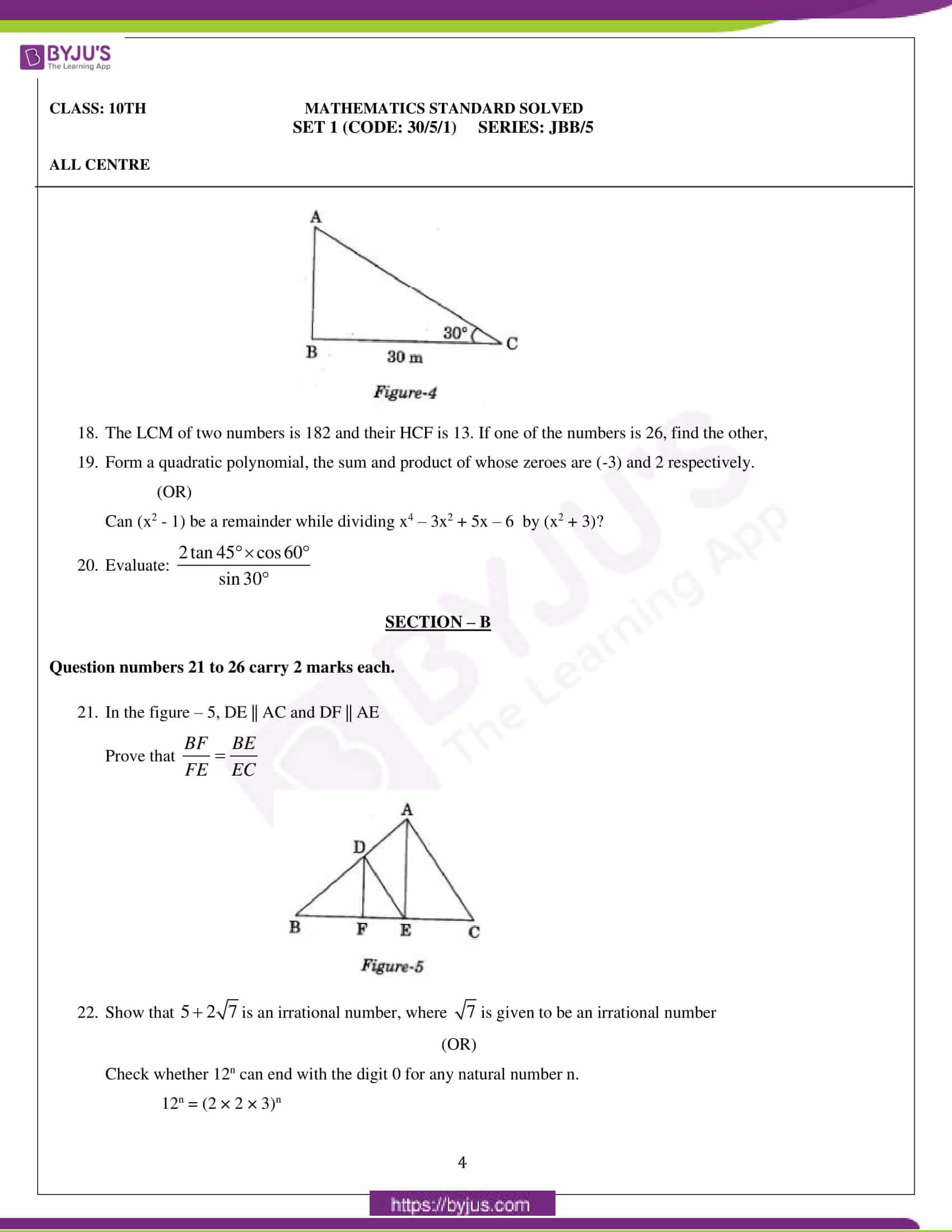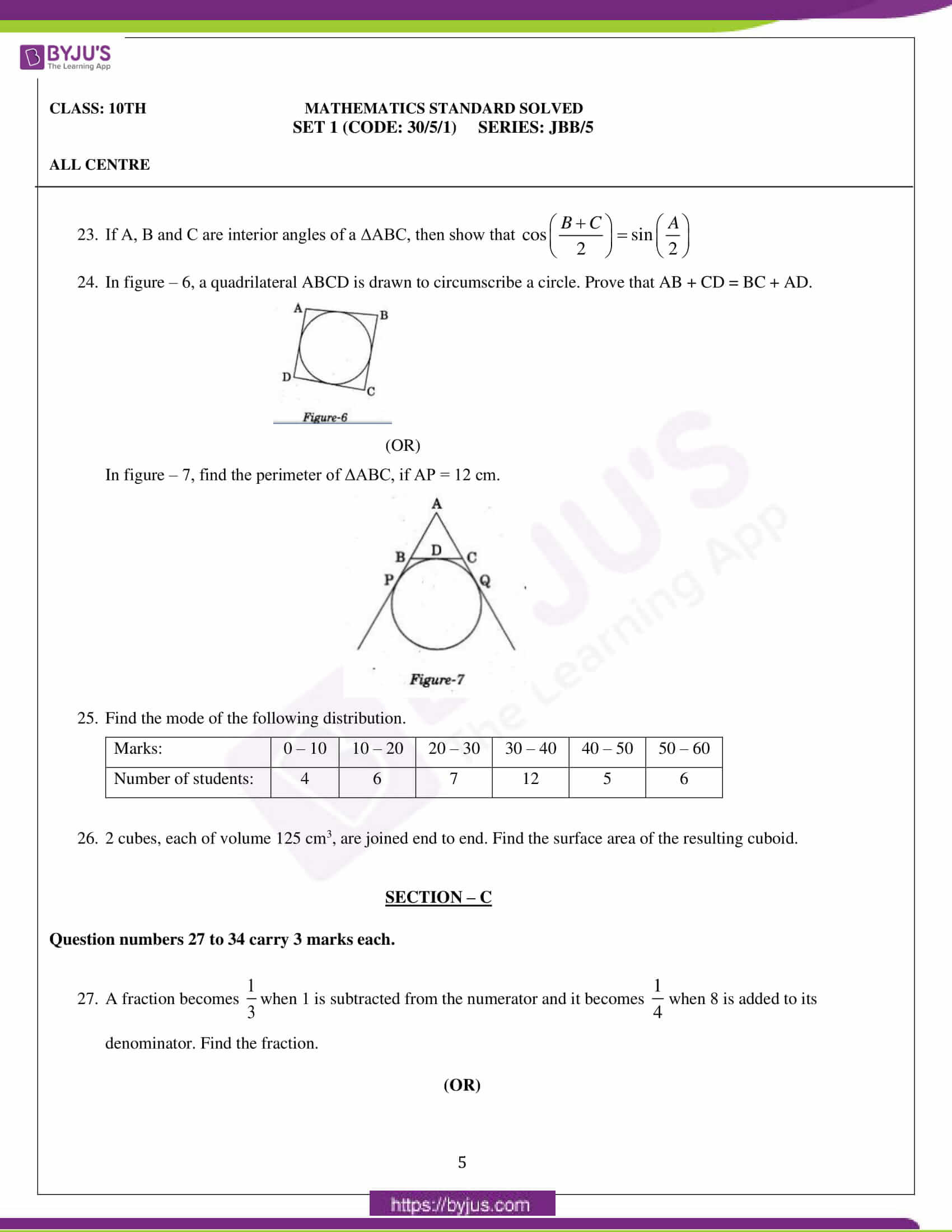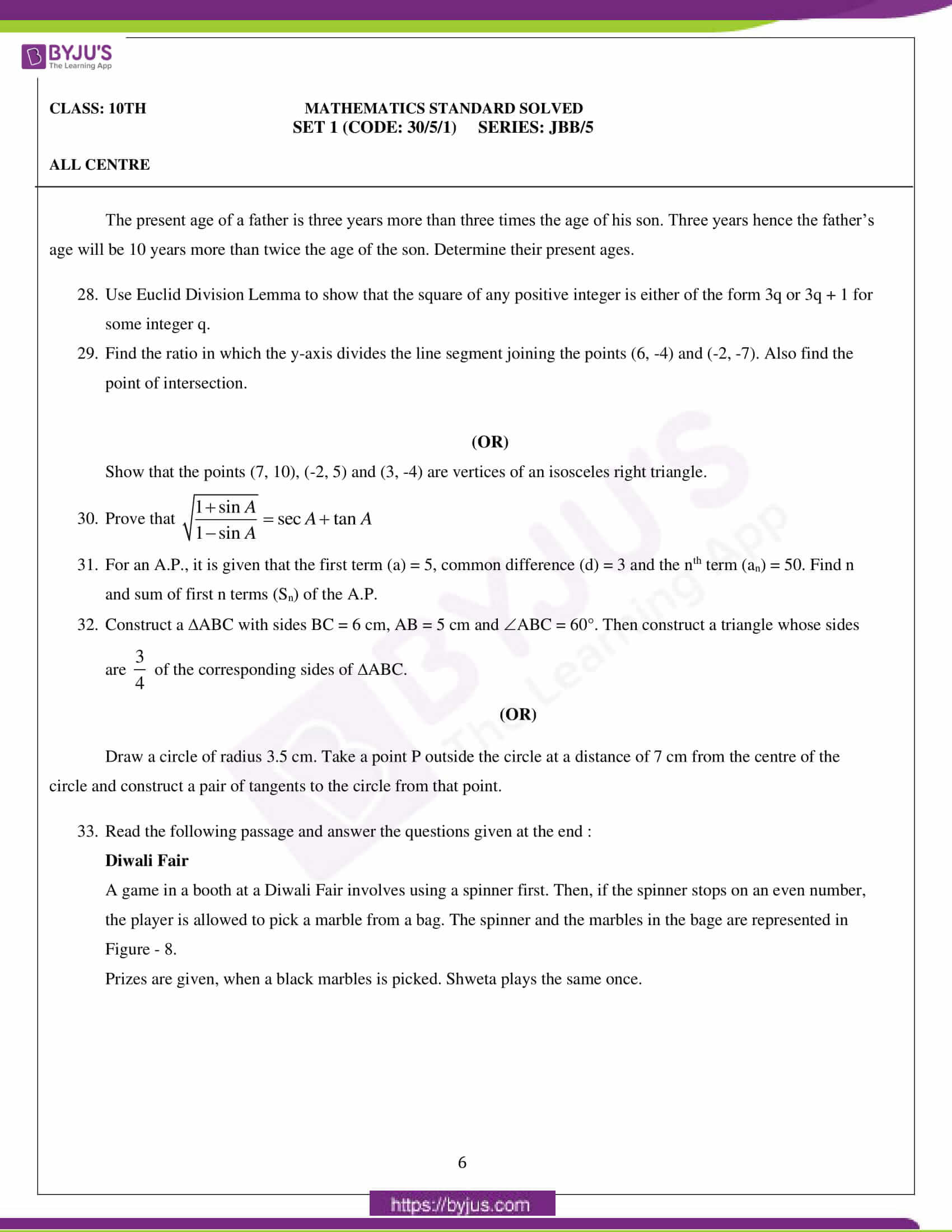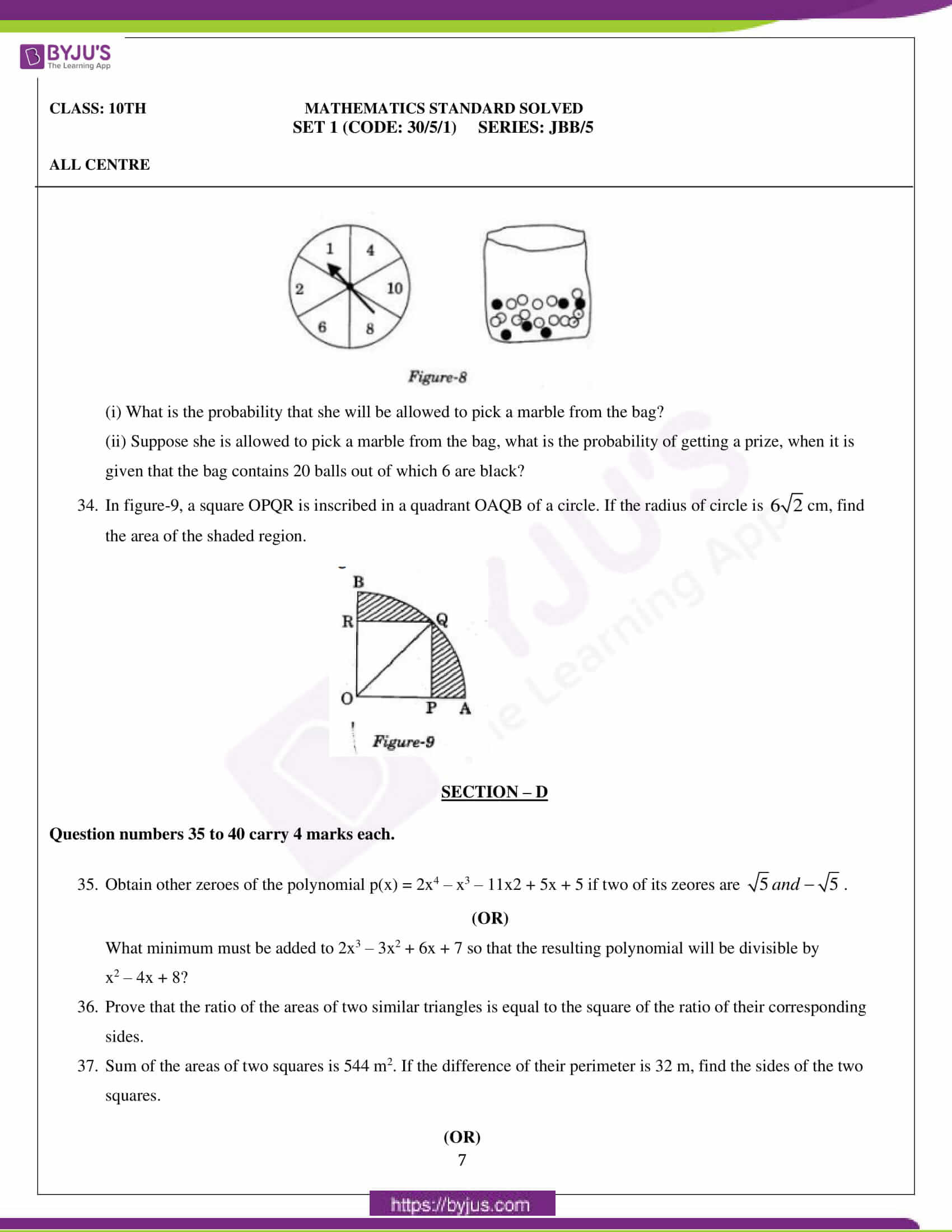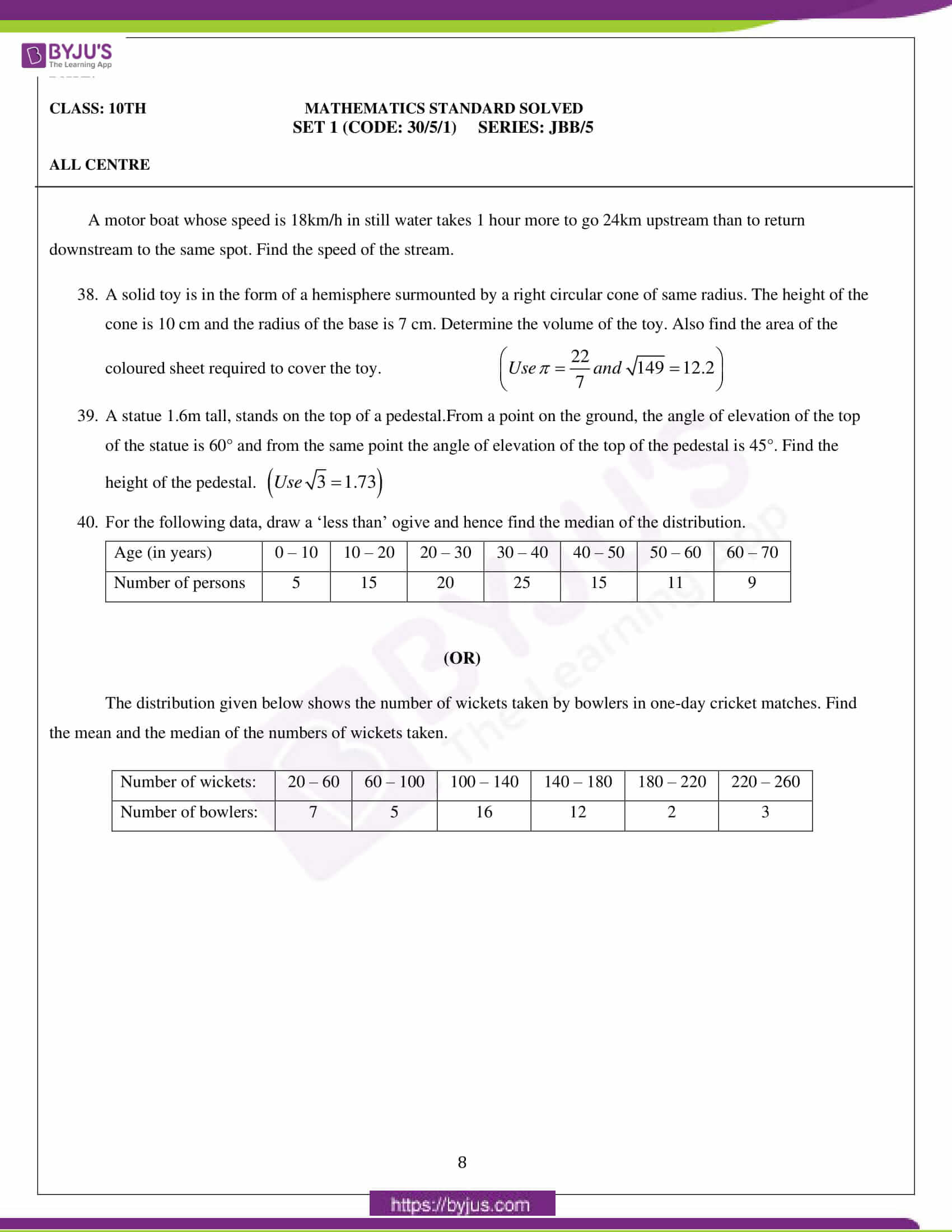### Mathematics Standard Set 1 (Code: 30/5/1, Series: JBB/5) Question Paper 2020

General Instructions:

1. This question paper comprises four sections – A, B, C and D. This question paper carries 40 questions. All questions are compulsory.
2. Section A: Question numbers 1 to 20 comprises 20 questions of one mark each.
3. Section B: Question numbers 21 to 26 comprises 6 questions of two marks each.
4. Section C: Question numbers 27 to 34 comprises 8 questions of three marks each.
5. Section D: Question numbers 35 to 40 comprises 6 questions of four marks each.
6. There is no overall choice in the question paper. However, an internal choice has been provided in 2 questions of one mark, 2 questions of two marks, 3 questions of three marks and 3 questions of four marks. You have to attempt only one of the choices in such questions.
7. In addition to this, separate instructions are given with each section and question, wherever necessary.
8. Use of calculators is not permitted.

#### SECTION – A

Question numbers 1 to 20 carry 1 mark each.

Question numbers 1 to 10 are multiple choice questions.

Choose the correct option.

1) On dividing a polynomial p(x) by x2 – 4, quotient and remainder are found to be x and 3 respectively. The polynomial p(x) is

(a) 3x2 + x – 12

(b) x3 – 4x + 3

(c) x2 + 3x – 4

(d) x3 – 4x – 3

2)In figure – 1, ABC is an isosceles triangle, right angled at C. Therefore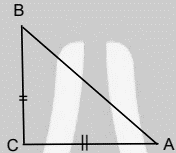Figure-1

(a) AB2 = 2AC2

(b) BC2 = 2AB2

(c) AC2 = 2AB2

(d) AB2 = 4AC2

3) The point on the x-axis which is equidistant from (-4, 0) and (10, 0) is

(a) (7, 0)

(b) (5, 0)

(c) (0, 0)

(d) (3, 0)

(OR)

The centre of a circle whose endpoints of a diameter are (-6, 3) and (6, 4) is

(a) (8, -1)

(b) (4, 7)

(c) $\left( 0,\frac{7}{2} \right)$

(d) $\left( 4,\frac{7}{2} \right)$

4) The value(s) of k for which the quadratic equation 2x2 + 5x + 2 = 0 has equal roots, is

(a) 4

(b) + 4

(c) – 4

(d) 0

5) Which of the following is not A.P.?

(a) – 1.2, 0.8, 2.8, ….. (b)$3,3+\sqrt{2},3+2\sqrt{2},3+3\sqrt{2}$

(c) $\frac{4}{3},\frac{7}{3},\frac{9}{3},\frac{12}{3},….$

(d) $\frac{-1}{5},\frac{-2}{5},\frac{-3}{5},….$

6) The pair of linear equations $\frac{3x}{2}+\frac{5y}{3}=7and\,9x+10y=14\,is$

(a) Consistent

(b) inconsistent

(c) Consistent with one solution

(d) consistent with many solutions

In figure – 2, PQ is tangent to the circle with centre at O, at the point B. If $\angle AOB=100{}^\circ ,then\angle ABP$is equal to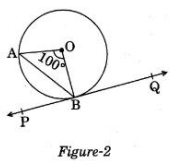(a) 50° (b) 40° (c) 60° (d) 80°

8) The radius of a sphere (in cm) whose volume is 12π cm3, is

(a) 3

(b) $3\sqrt{3}$

(c) ${{3}^{\frac{2}{3}}}$

(d) ${{3}^{\frac{1}{3}}}$

9) The distance between the points (m, -n) and (-m, n) is

(a) $\sqrt{{{m}^{2}}+{{n}^{2}}}$

(b) m + n

(c) $2\sqrt{{{m}^{2}}+{{n}^{2}}}$

(d) $\sqrt{2{{m}^{2}}+2{{n}^{2}}}$

10) In figure – 3, from an external point P, two tangents PQ and PR are drawn to a circle of radius 4 cm with centre O. If $\angle QPR=90{}^\circ ,$ then length of PQ is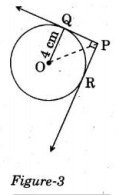(a) 3 cm

(b) 4 cm

(c) 2 cm

(d) $2\sqrt{2}\,cm$

Fill in the blanks in questions numbers 11 to 15.

11. The probability of an event that is sure to happen, is _______.

12. Simplest form of $\frac{1+{{\tan }^{2}}A}{1+{{\cot }^{2}}A}$ is ____________.

13. AOBC is a rectangle whose three vertices are A(0, -3), O(0, 0) and B(4, 0). The length of its diagonals is _________.

14. In the formula $\bar{x} = a+\left ( \frac{\sum f_{i}u_{i}}{\sum f_{i}} \right ) * h, u_{i}$= _______________ .

15. All concentric circles are ___________ to each other.

Answer the following question numbers 16 to 20

16. Find the sum of the first 100 natural numbers.

17. In figure – 4, the angle of elevation of the top of a tower from a point C on the ground, which is 30 m away from the foot of the tower, is 30°. Find the height of the tower.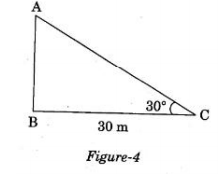18. The LCM of two numbers is 182 and their HCF is 13. If one of the numbers is 26, find the other number?

19. Form a quadratic polynomial, the sum and product of whose zeroes are (-3) and 2 respectively.

(OR)

Can (x2 – 1) be a remainder while dividing x4 – 3x2 + 5x – 6 by (x2 + 3)?

20) Evaluate:

$\frac{2\tan 45{}^\circ \times \cos 60{}^\circ }{\sin 30{}^\circ }$

#### SECTION – B

Question numbers 21 to 26 carry 2 marks each.

21. In the figure – 5, DE || AC and DF || AE

Prove that $\frac{BF}{FE}=\frac{BE}{EC}$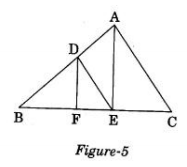22. Show that $5+2\sqrt{7}$is an irrational number, where $\sqrt{7}$is given to be an irrational number

(OR)

Check whether 12n can end with the digit 0 for any natural number n.

12n = (2 × 2 × 3)n

23. If A, B and C are interior angles of a ΔABC, then show that $\cos \left( \frac{B+C}{2} \right)=\sin \left( \frac{A}{2} \right)$

24. In figure – 6, a quadrilateral ABCD is drawn to circumscribe a circle. Prove that AB + CD = BC + AD.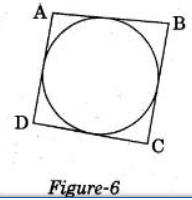(OR)

In figure – 7, find the perimeter of ΔABC, if AP = 12 cm.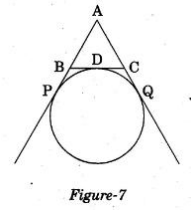25. Find the mode of the following distribution.

 Marks: 0 – 10 10 – 20 20 – 30 30 – 40 40 – 50 50 – 60 Number of students: 4 6 7 12 5 6

26. 2 cubes, each of volume 125 cm3, are joined end to end. Find the surface area of the resulting cuboid.

#### SECTION – C

Question numbers 27 to 34 carry 3 marks each.

27. A fraction becomes $\frac{1}{3}$when 1 is subtracted from the numerator and it becomes $\frac{1}{4}$when 8 is added to its denominator. Find the fraction.

(OR)

The present age of a father is three years more than three times the age of his son. Three years hence the father’s age will be 10 years more than twice the age of the son. Determine their present ages.

28. Use the Euclid Division Lemma to show that the square of any positive integer is either of the form 3q or 3q + 1 for some integer q.

29. Find the ratio in which the y-axis divides the line segment joining the points (6, -4) and (-2, -7). Also find the point of intersection.

(OR)

Show that the points (7, 10), (-2, 5) and (3, -4) are vertices of an isosceles right triangle.

30. Prove that $\sqrt{\frac{1+\sin A}{1-\sin A}}=\sec A+\tan A$

31. For an A.P., it is given that the first term (a) = 5, common difference (d) = 3 and the nth term (an) = 50. Find n and sum of first n terms (Sn) of the A.P.

32. Construct a ΔABC with sides BC = 6 cm, AB = 5 cm and ∠ABC = 60°. Then construct a triangle whose sides are $\frac{3}{4}$ of the corresponding sides of ΔABC.

(OR)

Draw a circle of radius 3.5 cm. Take a point P outside the circle at a distance of 7 cm from the centre of the circle and construct a pair of tangents to the circle from that point.

33. Read the following passage and answer the questions given at the end:

Diwali Fair

A game in a booth at a Diwali Fair involves using a spinner first. Then, if the spinner stops on an even number, the player is allowed to pick a marble from a bag. The spinner and the marbles in the bage are represented in Figure – 8.

Prizes are given, when a black marble is picked. Shweta plays the same once.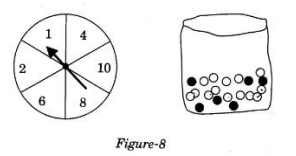(i) What is the probability that she will be allowed to pick a marble from the bag?

(ii) Suppose she is allowed to pick a marble from the bag, what is the probability of getting a prize when it is given that the bag contains 20 balls out of which 6 are black?

In figure-9, a square OPQR is inscribed in a quadrant OAQB of a circle. If the radius of the circle is $6\sqrt{2}$cm, find the area of the shaded region.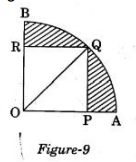#### SECTION – D

Question numbers 35 to 40 carry 4 marks each.

35. Obtain other zeroes of the polynomial p(x) = 2x4 – x3 – 11×2 + 5x + 5 if two of its zeros are $\sqrt{5}\,and\,-\sqrt{5}$.

(OR)

What minimum must be added to 2x3 – 3x2 + 6x + 7 so that the resulting polynomial will be divisible by x2 – 4x + 8?

36. Prove that the ratio of the areas of two similar triangles is equal to the square of the ratio of their corresponding sides.

37. Sum of the areas of two squares is 544 m2. If the difference of their perimeter is 32 m, find the sides of the two squares.

(OR)

A motor boat whose speed is 18km/h in still water takes 1 hour more to go 24km upstream than to return downstream to the same spot. Find the speed of the stream.

38. A solid toy is in the form of a hemisphere surmounted by a right circular cone of the same radius. The height of the cone is 10 cm and the radius of the base is 7 cm. Determine the volume of the toy. Also find the area of the coloured sheet required to cover the toy.

$\left( Use\,\pi =\frac{22}{7}\,and\,\sqrt{149}=12.2 \right)$

39. A statue 1.6m tall, stands on the top of a pedestal.From a point on the ground, the angle of elevation of the top of the statue is 60° and from the same point the angle of elevation of the top of the pedestal is 45°. Find the height of the pedestal.

$\left( Use\,\sqrt{3}=1.73 \right)$

40. For the following data, draw a ‘less than’ ogive and hence find the median of the distribution.

 Age (in years) 0 – 10 10 – 20 20 – 30 30 – 40 40 – 50 50 – 60 60 – 70 Number of persons 5 15 20 25 15 11 9

(OR)

The distribution given below shows the number of wickets taken by bowlers in one-day cricket matches. Find the mean and the median of the numbers of wickets taken.

 Number of wickets: 20 – 60 60 – 100 100 – 140 140 – 180 180 – 220 220 – 260 Number of bowlers: 7 5 16 12 2 3

## CBSE Class 10 Maths Standard Question Paper 2020 Set 2 (30/5/2)

Students can have a look at the CBSE Class 10 Mathematics Standard Set 2 Question Paper 2020 below: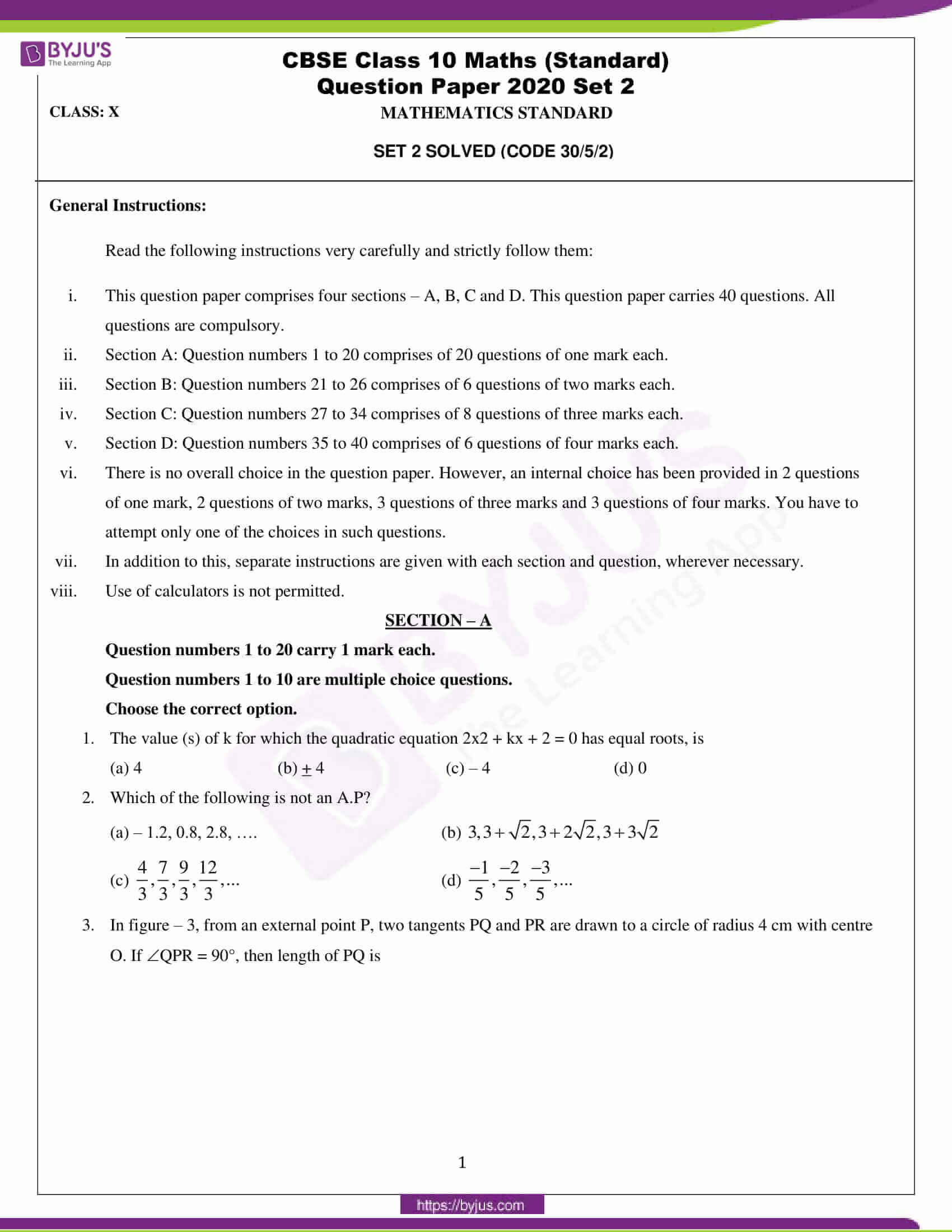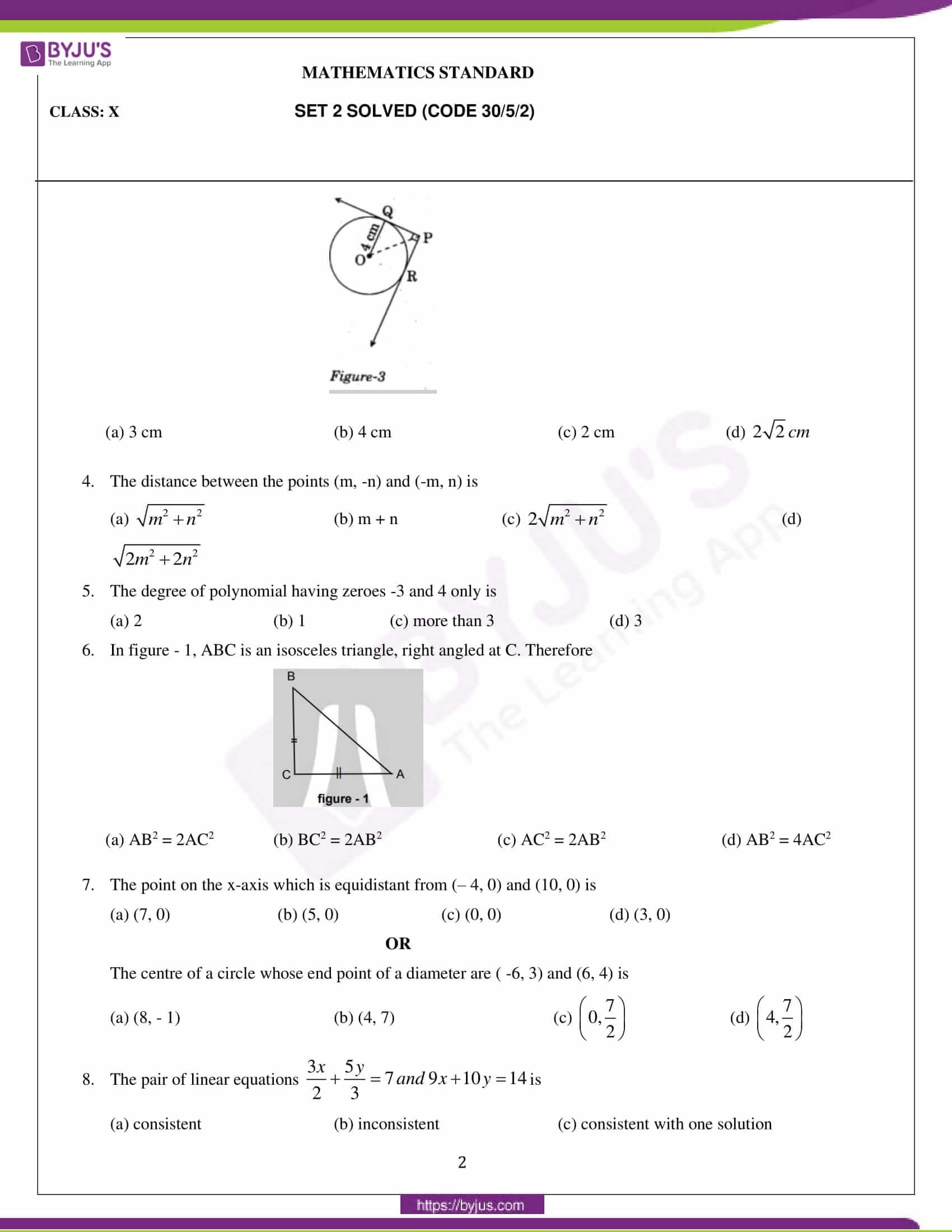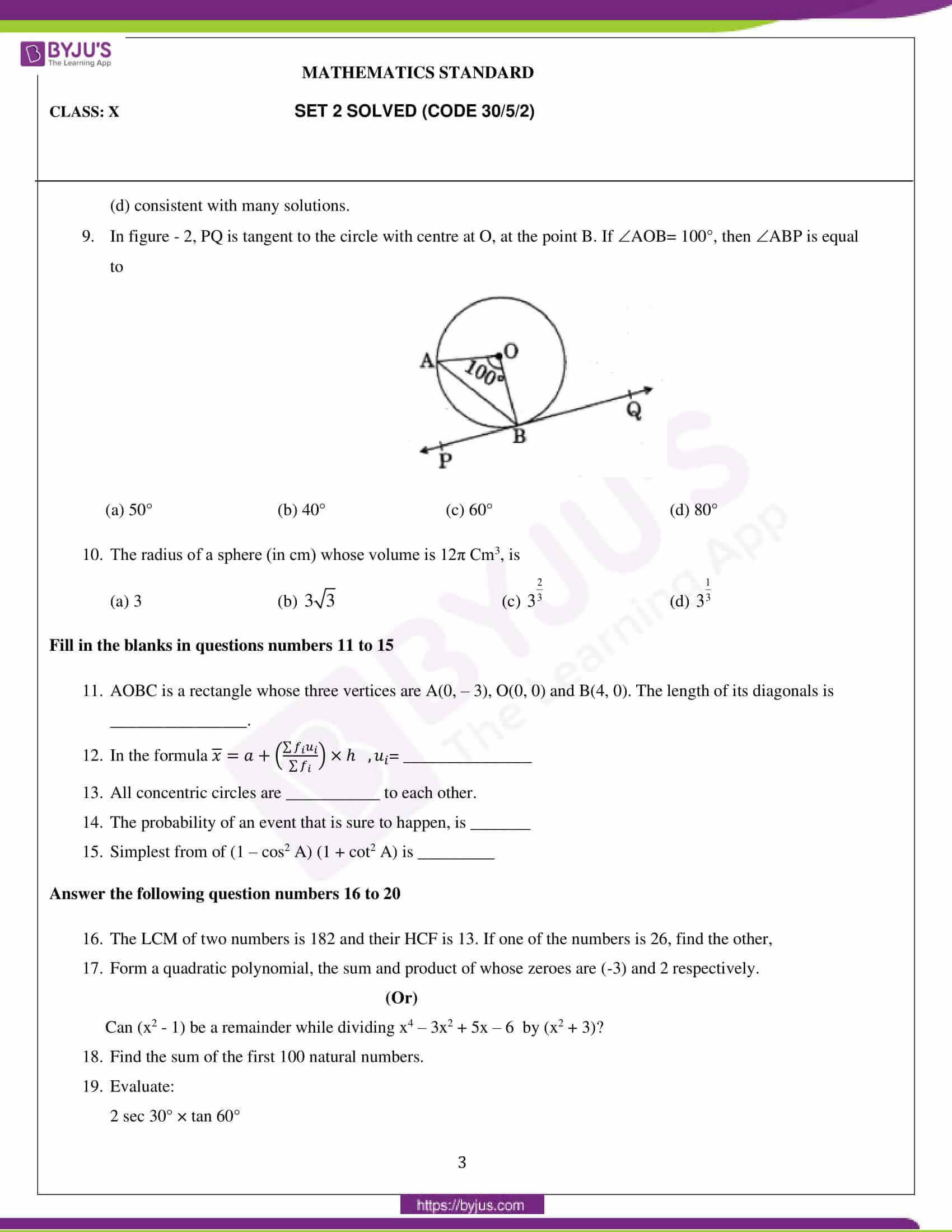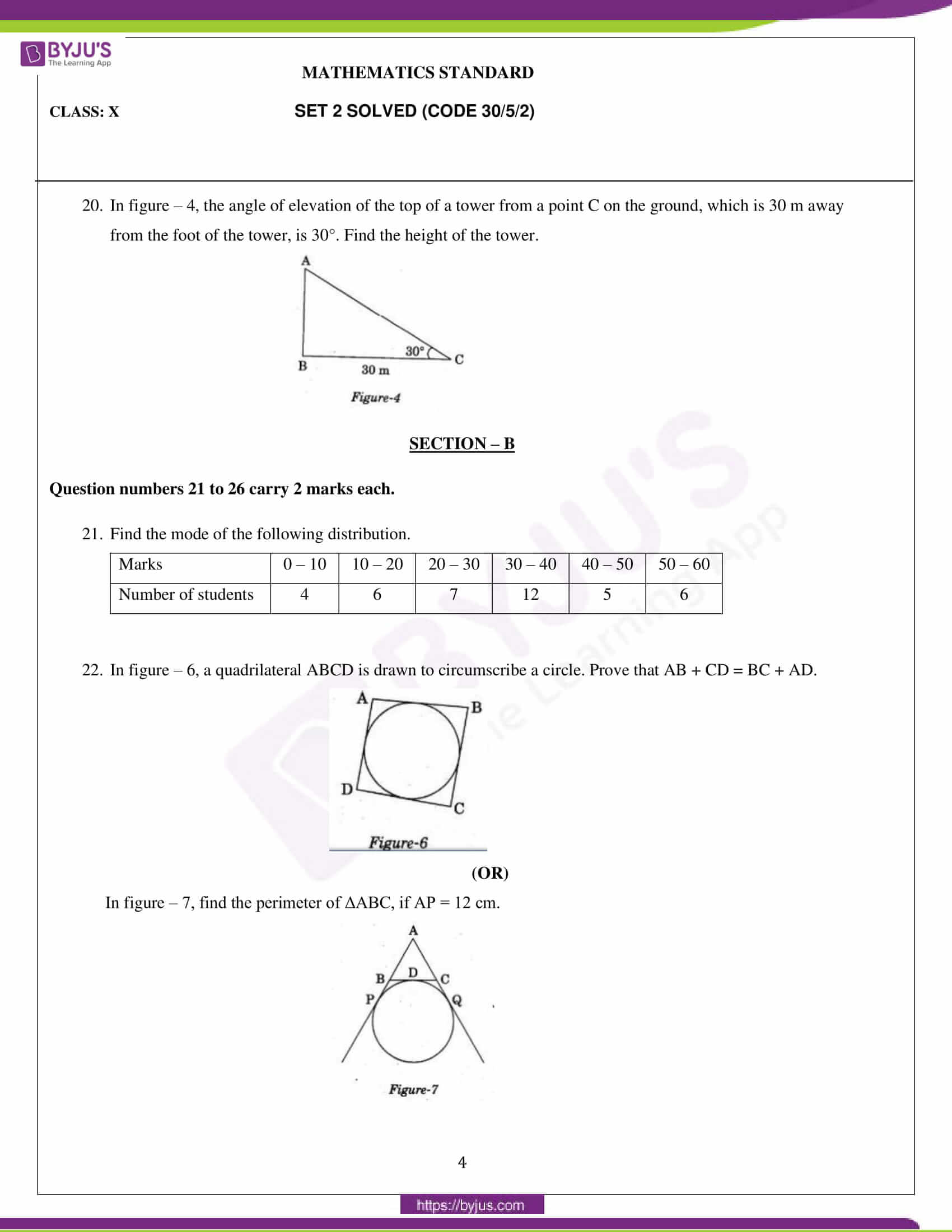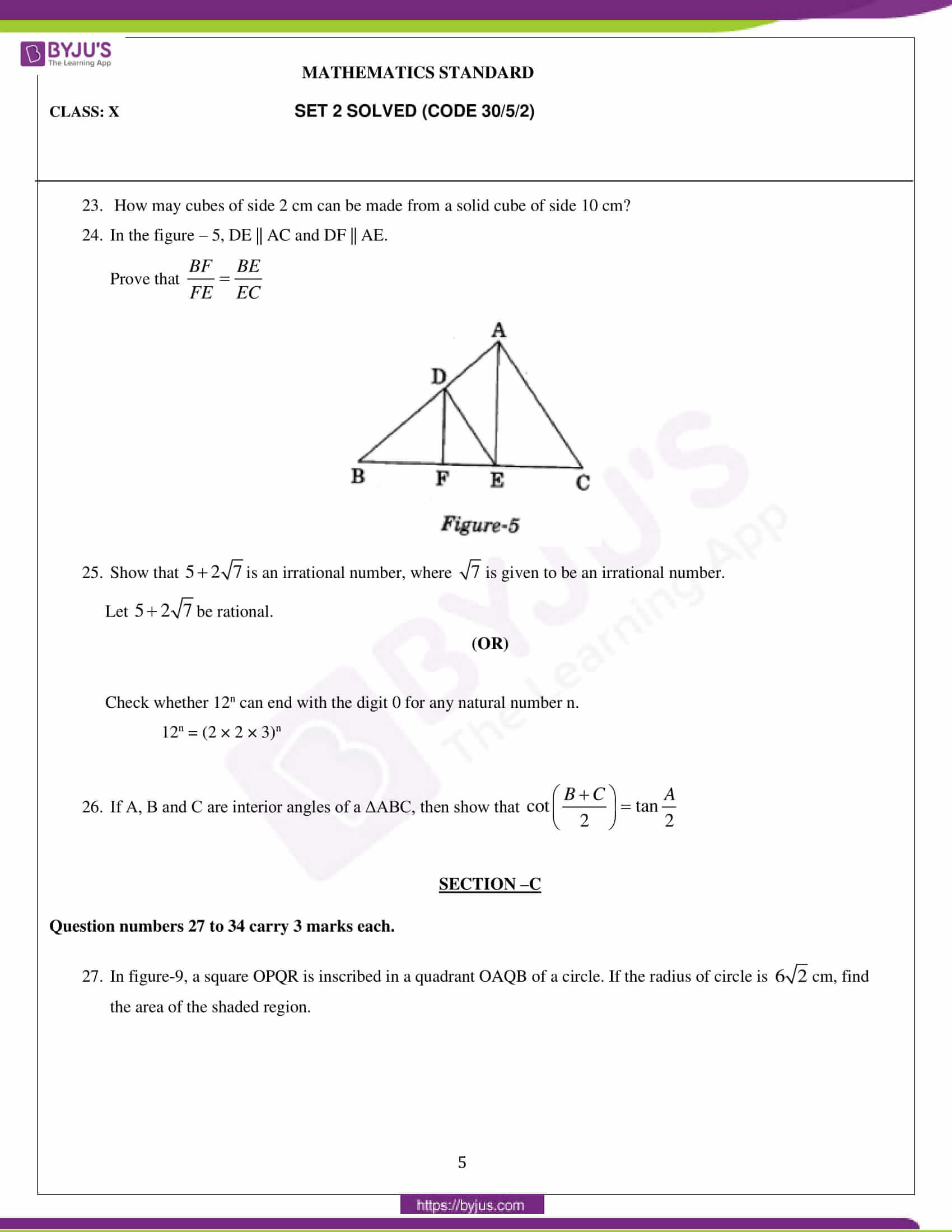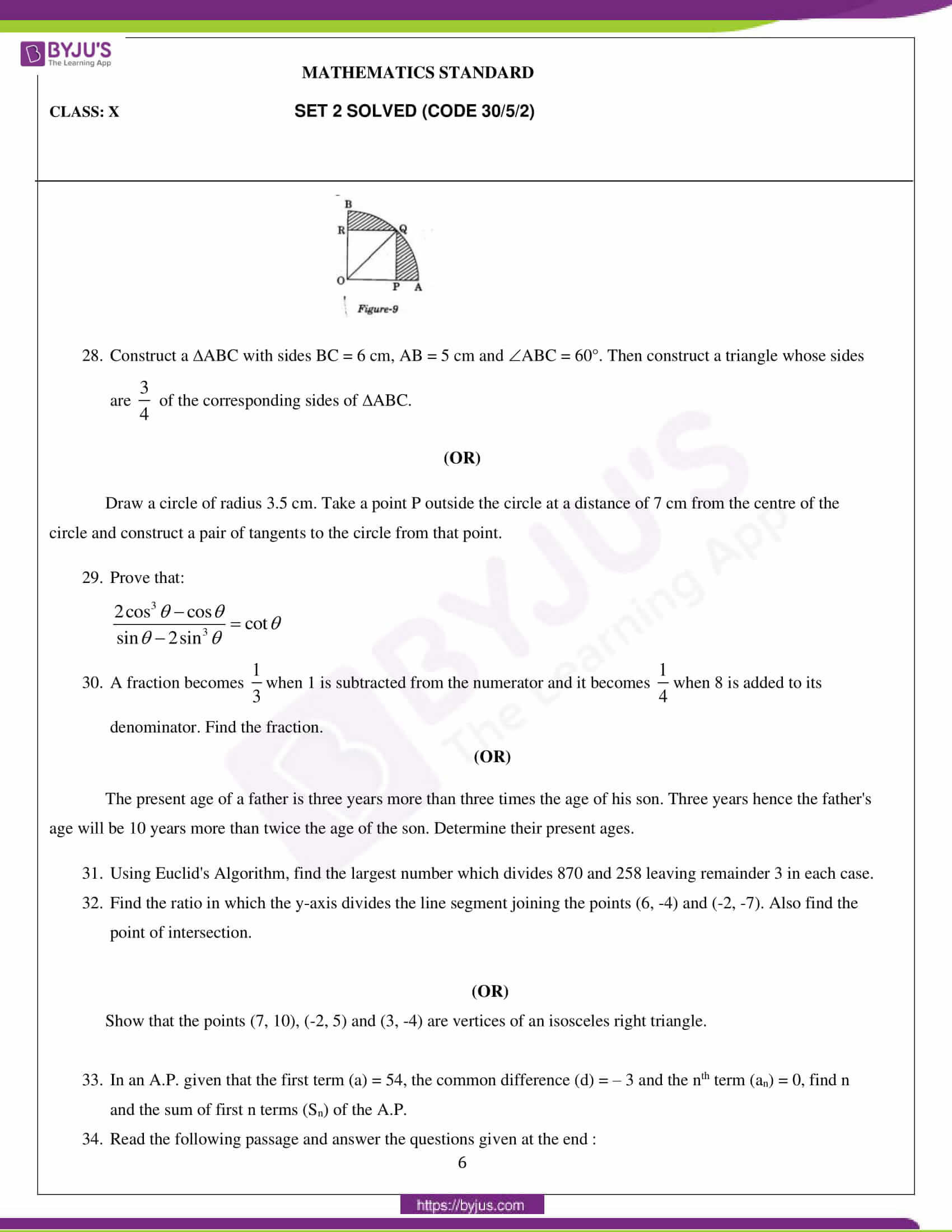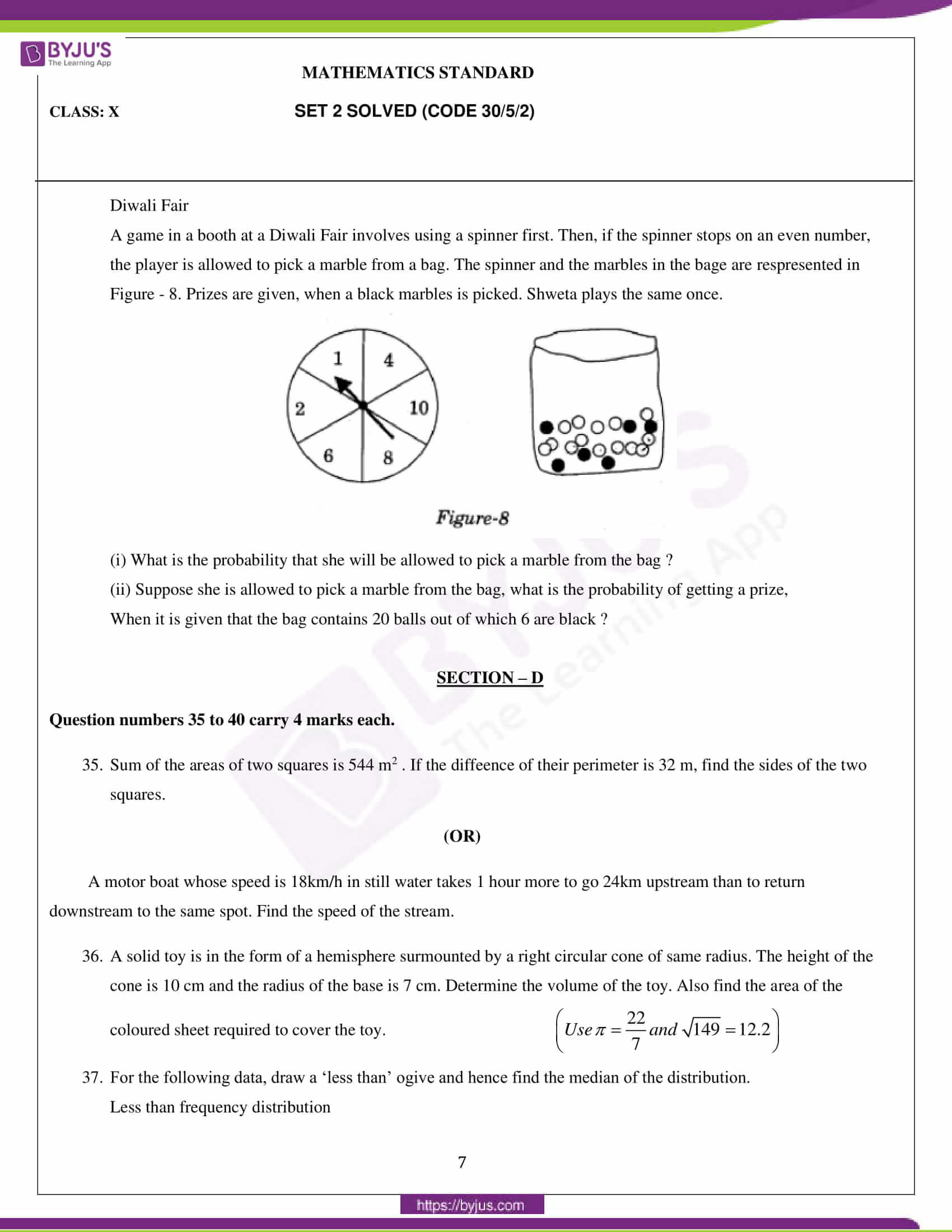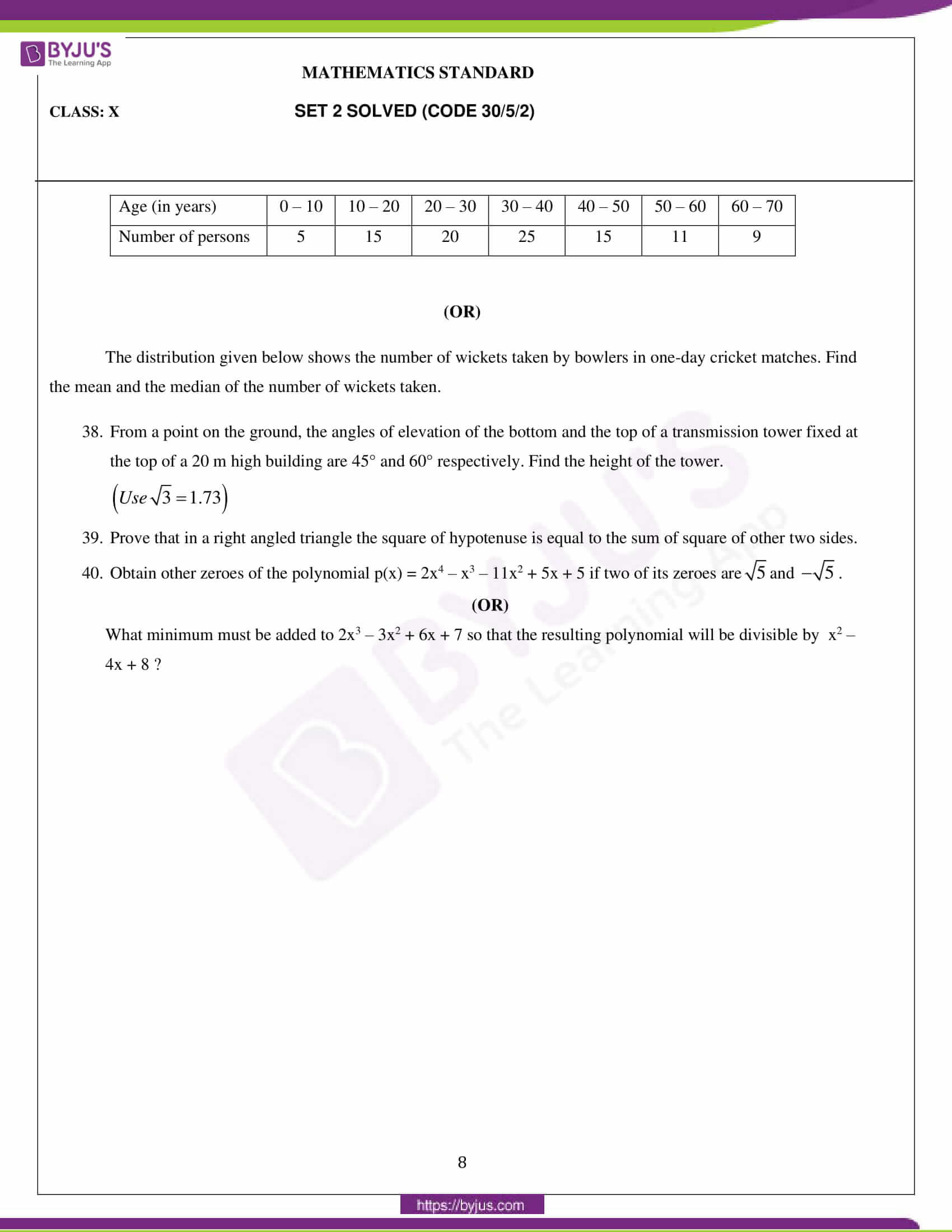### Mathematics Standard Set 2 (Code: 30/5/2) Question Paper 2020

General Instructions:

1. This question paper comprises four sections – A, B, C and D. This question paper carries 40 questions. All questions are compulsory.
2. Section A: Question numbers 1 to 20 comprises 20 questions of one mark each.
3. Section B: Question numbers 21 to 26 comprises 6 questions of two marks each.
4. Section C: Question numbers 27 to 34 comprises 8 questions of three marks each.
5. Section D: Question numbers 35 to 40 comprises 6 questions of four marks each.
6. There is no overall choice in the question paper. However, an internal choice has been provided in 2 questions of one mark, 2 questions of two marks, 3 questions of three marks and 3 questions of four marks. You have to attempt only one of the choices in such questions.
7. In addition to this, separate instructions are given with each section and question, wherever necessary.
8. Use of calculators is not permitted.

#### SECTION – A

Question numbers 1 to 20 carry 1 mark each.

Question numbers 1 to 10 are multiple choice questions.

Choose the correct option.

1. The value (s) of k for which the quadratic equation 2x2 + kx + 2 = 0 has equal roots, is

(a) 4

(b) + 4

(c) – 4

(d) 0

2. Which of the following is not an A.P?

(a) – 1.2, 0.8, 2.8, ….

(b) $3,3+\sqrt{2},3+2\sqrt{2},3+3\sqrt{2}$

(c) $\frac{4}{3},\frac{7}{3},\frac{9}{3},\frac{12}{3},…$

(d) $\frac{-1}{5},\frac{-2}{5},\frac{-3}{5},…$

3. In figure – 3, from an external point P, two tangents PQ and PR are drawn to a circle of radius 4 cm with centre O. If ∠QPR = 90°, then length of PQ is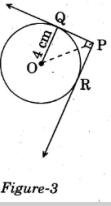(a) 3 cm

(b) 4 cm

(c) 2 cm

(d) $2\sqrt{2}\,cm$

4. The distance between the points (m, -n) and (-m, n) is

(a) $\sqrt{{{m}^{2}}+{{n}^{2}}}$

(b) m + n

(c) $2\sqrt{{{m}^{2}}+{{n}^{2}}}$$2\sqrt{{{m}^{2}}+{{n}^{2}}}$

(d) $\sqrt{2{{m}^{2}}+2{{n}^{2}}}$$\sqrt{2{{m}^{2}}+2{{n}^{2}}}$

5. The degree of polynomial having zeros -3 and 4 only is

(a) 2

(b) 1

(c) more than 3

(d) 3

6. In figure – 1, ABC is an isosceles triangle, right angled at C. Therefore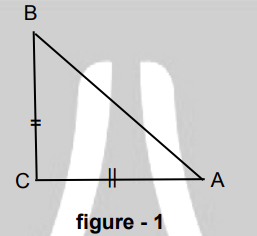(a) AB2 = 2AC2

(b) BC2 = 2AB2

(c) AC2 = 2AB2

(d) AB2 = 4AC2

7. The point on the x-axis which is equidistant from (– 4, 0) and (10, 0) is

(a) (7, 0)

(b) (5, 0)

(c) (0, 0)

(d) (3, 0)

OR

The centre of a circle whose end point of a diameter are ( -6, 3) and (6, 4) is

(a) (8, – 1)

(b) (4, 7)

(c) $\left( 0,\frac{7}{2} \right)$

(d) $\left( 4,\frac{7}{2} \right)$

8. The pair of linear equations is

$\frac{3x}{2}+\frac{5y}{3}=7\,and\,9x+10y=14$

(a) consistent

(b) inconsistent

(c) consistent with one solution

(d) consistent with many solutions

9. In figure – 2, PQ is tangent to the circle with centre at O, at the point B. If ∠AOB= 100°, then ∠ABP is equal to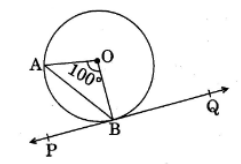(a) 50°

(b) 40°

(c) 60°

(d) 80°

10. The radius of a sphere (in cm) whose volume is 12π Cm3, is

(a) 3

(b) $3\sqrt{3}$

(c) ${{3}^{\frac{2}{3}}}$

(d) ${{3}^{\frac{1}{3}}}$

Fill in the blanks in questions numbers 11 to 15.

11. AOBC is a rectangle whose three vertices are A(0, – 3), O(0, 0) and B(4, 0). The length of its diagonals is ________________.

12. In the formula $\bar{x} = a+\left ( \frac{\sum f_{i}u_{i}}{\sum f_{i}} \right ) * h, u_{i}$ = _______________

13. All concentric circles are ___________ to each other.

14. The probability of an event that is sure to happen, is _______.

15. Simplest form of (1 – cos2 A) (1 + cot2 A) is _________.

Answer the following question numbers 16 to 20

16. The LCM of two numbers is 182 and their HCF is 13. If one of the numbers is 26, find the other number?

17. Form a quadratic polynomial, the sum and product of whose zeroes are (-3) and 2 respectively.

OR

Can (x2 – 1) be a remainder while dividing x4 – 3x2 + 5x – 6 by (x2 + 3)?

18. Find the sum of the first 100 natural numbers.

19. Evaluate:

2 sec 30° × tan 60°

20. In figure – 4, the angle of elevation of the top of a tower from a point C on the ground, which is 30 m away from the foot of the tower, is 30°. Find the height of the tower.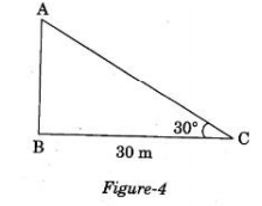#### SECTION – B

Question numbers 21 to 26 carry 2 marks each.

21. Find the mode of the following distribution.

 Marks 0 – 10 10 – 20 20 – 30 30 – 40 40 – 50 50 – 60 Number of students 4 6 7 12 5 6

22. In figure – 6, a quadrilateral ABCD is drawn to circumscribe a circle. Prove that AB + CD = BC + AD.(OR)

In figure – 7, find the perimeter of ΔABC, if AP = 12 cm.23. How many cubes of side 2 cm can be made from a solid cube of side 10 cm?

24. In the figure – 5, DE || AC and DF || AE.

Prove that $\frac{BF}{FE}=\frac{BE}{EC}$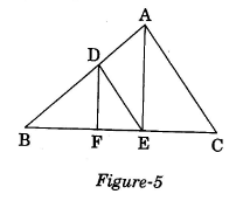25. Show that $5+2\sqrt{7}$is an irrational number, where $\sqrt{7}$$\sqrt{7}$is given to be an irrational number.

Let $5+2\sqrt{7}$be rational.

(OR)

Check whether 12n can end with the digit 0 for any natural number n.

12n = (2 × 2 × 3)n

26. If A, B and C are interior angles of a ΔABC, then show that

$\cot \left( \frac{B+C}{2} \right)=\tan \frac{A}{2}$

#### SECTION – C

Question numbers 27 to 34 carry 3 marks each.

27. In figure-9, a square OPQR is inscribed in a quadrant OAQB of a circle. If the radius of the circle is $6\sqrt{2}$cm, find the area of the shaded region.28. Construct a ΔABC with sides BC = 6 cm, AB = 5 cm and ∠ABC = 60°. Then construct a triangle whose sides are $\frac{3}{4}$ of the corresponding sides of ΔABC.

(OR)

Draw a circle of radius 3.5 cm. Take a point P outside the circle at a distance of 7 cm from the centre of the circle and construct a pair of tangents to the circle from that point.

29. Prove that:

$\frac{2{{\cos }^{3}}\theta -\cos \theta }{\sin \theta -2{{\sin }^{3}}\theta }=\cot \theta$

30. A fraction becomes $\frac{1}{3}$when 1 is subtracted from the numerator and it becomes $\frac{1}{4}$when 8 is added to its denominator. Find the fraction.

(OR)

The present age of a father is three years more than three times the age of his son. Three years hence the father’s age will be 10 years more than twice the age of the son. Determine their present ages.

31. Using Euclid’s Algorithm, find the largest number which divides 870 and 258 leaving remainder 3 in each case.

32. Find the ratio in which the y-axis divides the line segment joining the points (6, -4) and (-2, -7). Also find the point of intersection.

(OR)

Show that the points (7, 10), (-2, 5) and (3, -4) are vertices of an isosceles right triangle.

33. In an A.P. given that the first term (a) = 54, the common difference (d) = – 3 and the nth term (an) = 0, find n and the sum of first n terms (Sn) of the A.P.

34. Read the following passage and answer the questions given at the end:

Diwali Fair

A game in a booth at a Diwali Fair involves using a spinner first. Then, if the spinner stops on an even number, the player is allowed to pick a marble from a bag. The spinner and the marbles in the bag are represented in Figure – 8. Prizes are given, when a black marble is picked. Shweta plays the same once.(i) What is the probability that she will be allowed to pick a marble from the bag ?

(ii) Suppose she is allowed to pick a marble from the bag, what is the probability of getting a prize, when it is given that the bag contains 20 balls out of which 6 are black?

#### SECTION – D

Question numbers 35 to 40 carry 4 marks each.

35. Sum of the areas of two squares is 544 m2. If the difference of their perimeter is 32 m, find the sides of the two squares.

(OR)

A motor boat whose speed is 18km/h in still water takes 1 hour more to go 24km upstream than to return downstream to the same spot. Find the speed of the stream.

36. A solid toy is in the form of a hemisphere surmounted by a right circular cone of the same radius. The height of the cone is 10 cm and the radius of the base is 7 cm. Determine the volume of the toy. Also find the area of the coloured sheet required to cover the toy.

$\left( Use\,\pi =\frac{22}{7}\,and\,\sqrt{149}=12.2 \right)$

37. For the following data, draw a ‘less than’ ogive and hence find the median of the distribution.

Less than frequency distribution

 Age (in years) 0 – 10 10 – 20 20 – 30 30 – 40 40 – 50 50 – 60 60 – 70 Number of persons 5 15 20 25 15 11 9

(OR)

The distribution given below shows the number of wickets taken by bowlers in one-day cricket matches. Find the mean and the median of the number of wickets taken.

38. From a point on the ground, the angles of elevation of the bottom and the top of a transmission tower fixed at the top of a 20 m high building are 45° and 60° respectively. Find the height of the tower.

$\left( Use\,\sqrt{3}=1.73 \right)$

39. Prove that in a right angled triangle the square of hypotenuse is equal to the sum of squares of the other two sides.

40. Obtain other zeroes of the polynomial p(x) = 2x4 – x3 – 11x2 + 5x + 5 if two of its zeroes are$\sqrt{5}$and $-\sqrt{5}$.

(OR)

What minimum must be added to 2x3 – 3x2 + 6x + 7 so that the resulting polynomial will be divisible by x2 – 4x + 8 ?

## CBSE Class 10 Mathematics Question Paper 2020 Set 3 (30/5/3)

Students can have a look at the CBSE Class 10 Maths Question Paper 2020 Set 3 (paper code 30/5/3) below: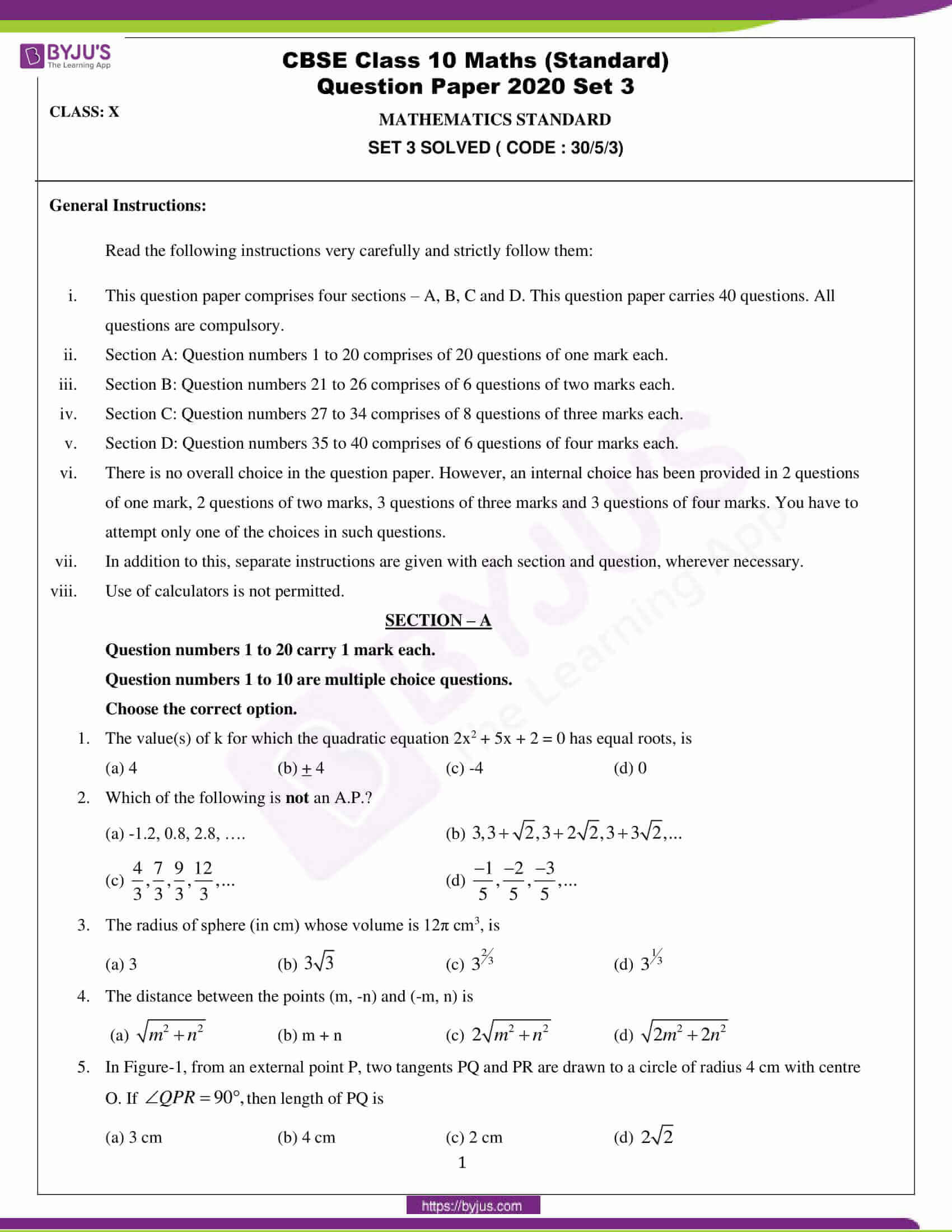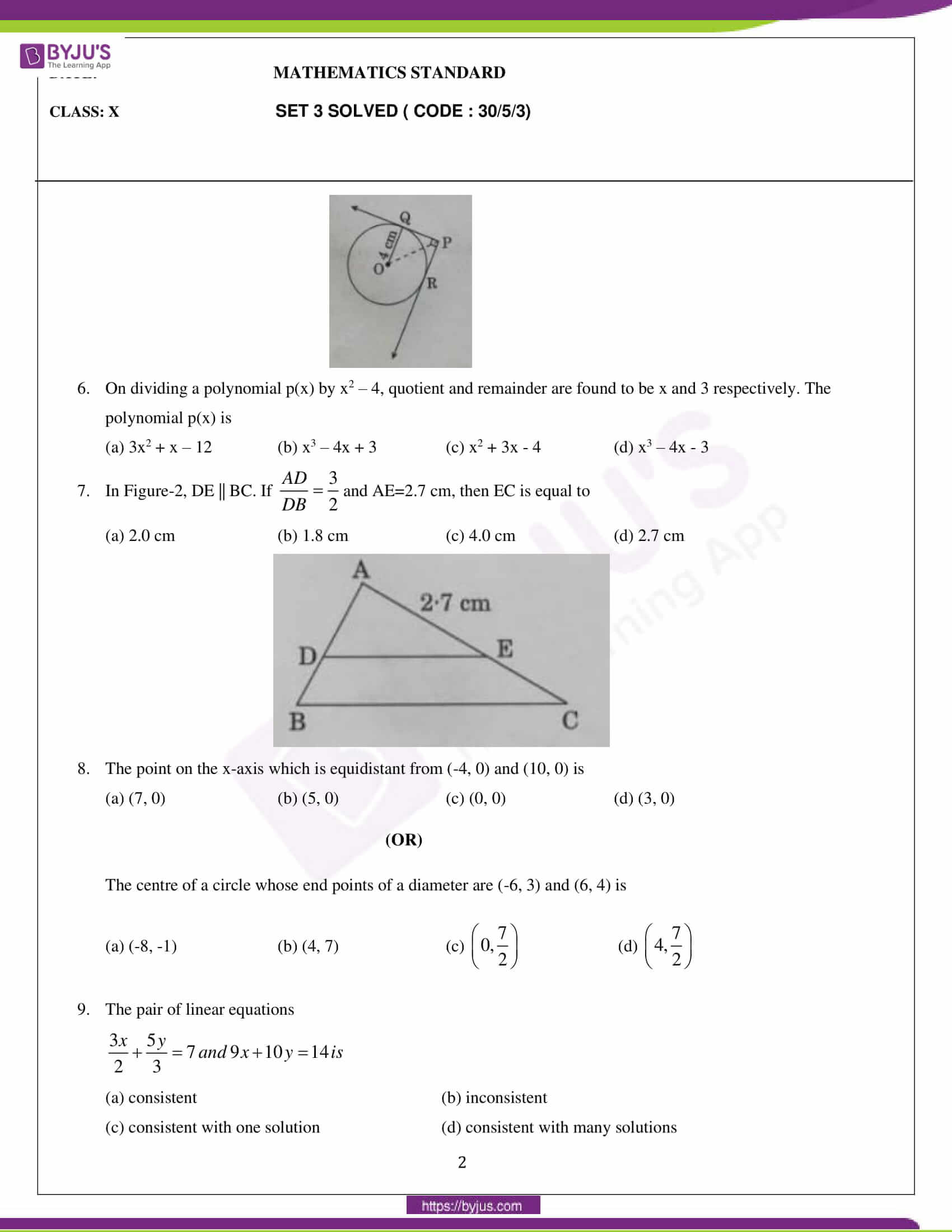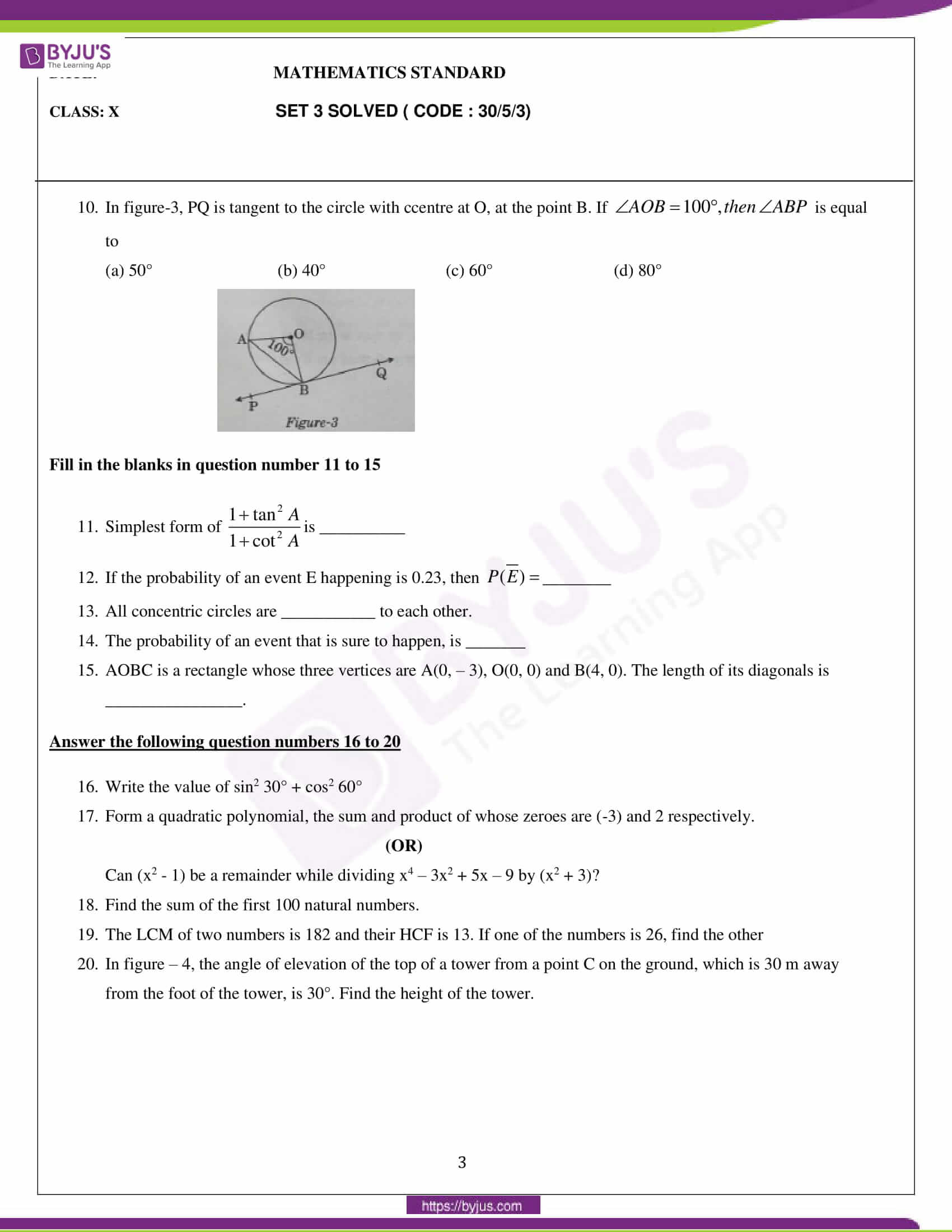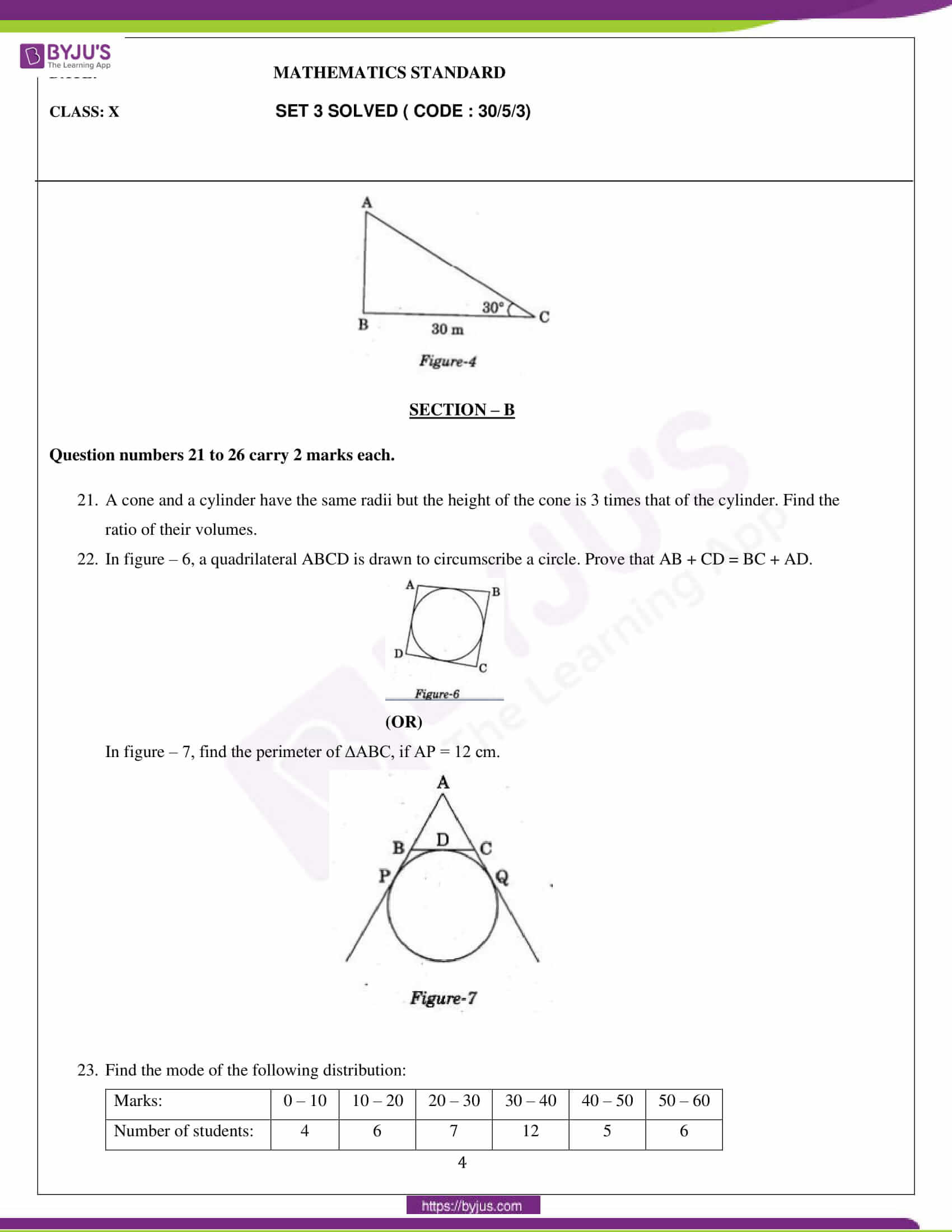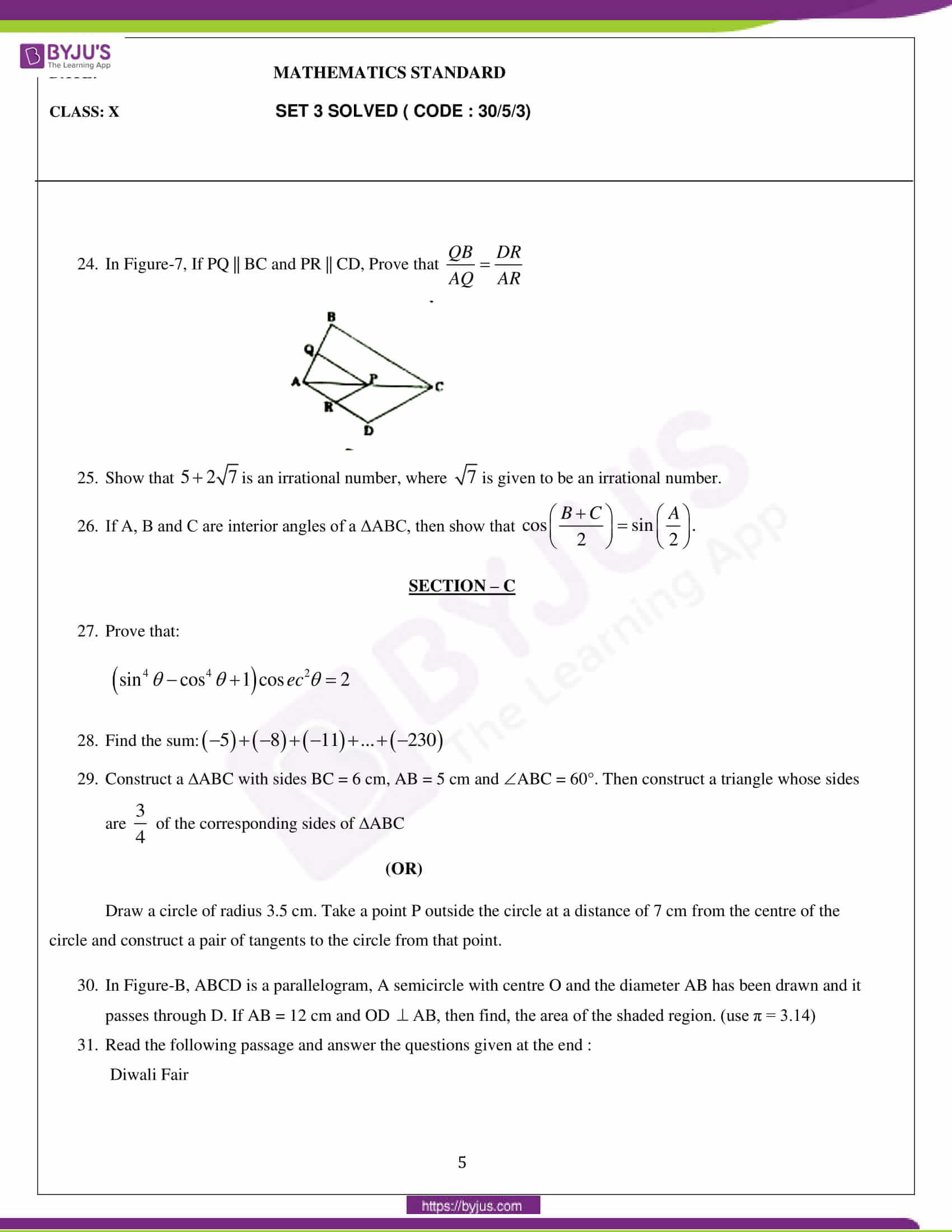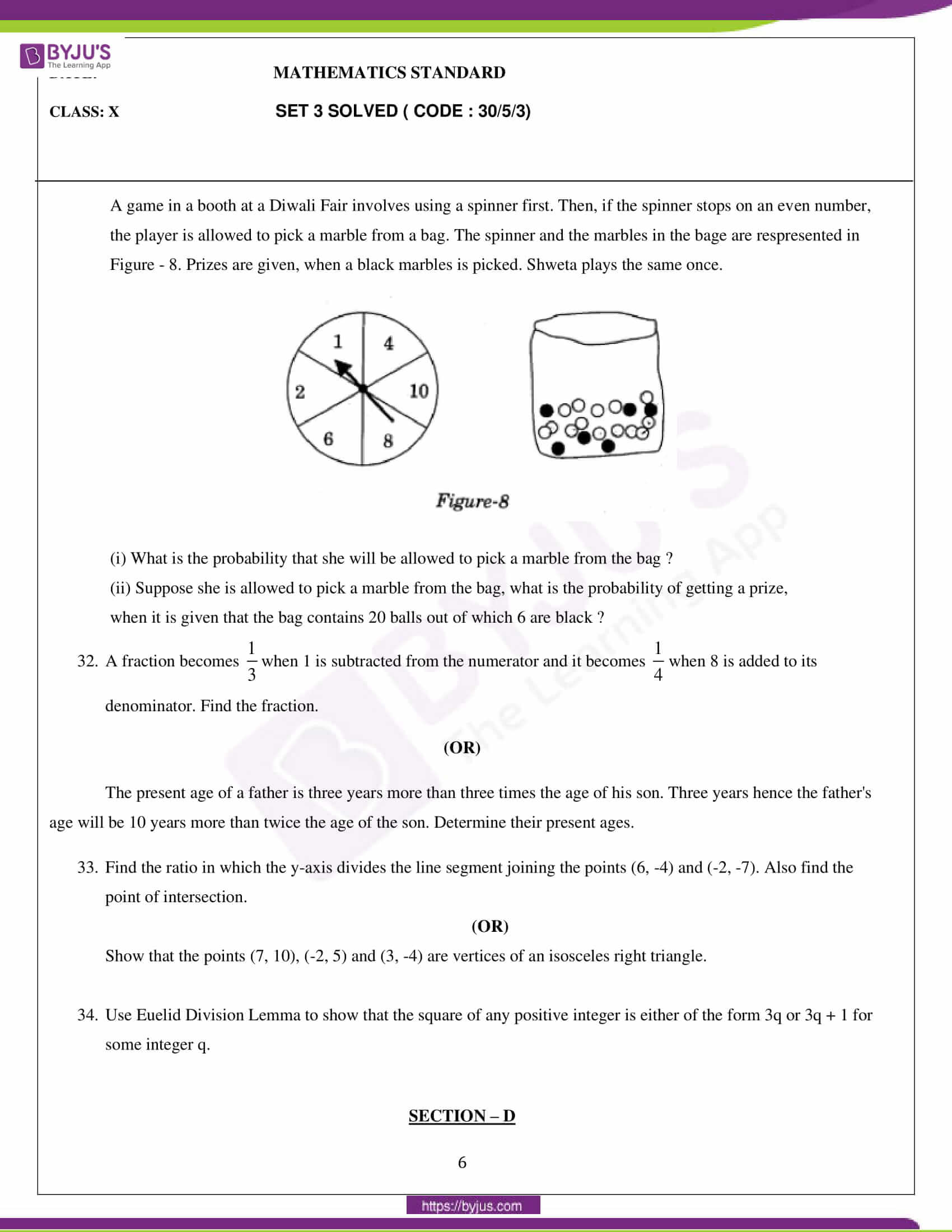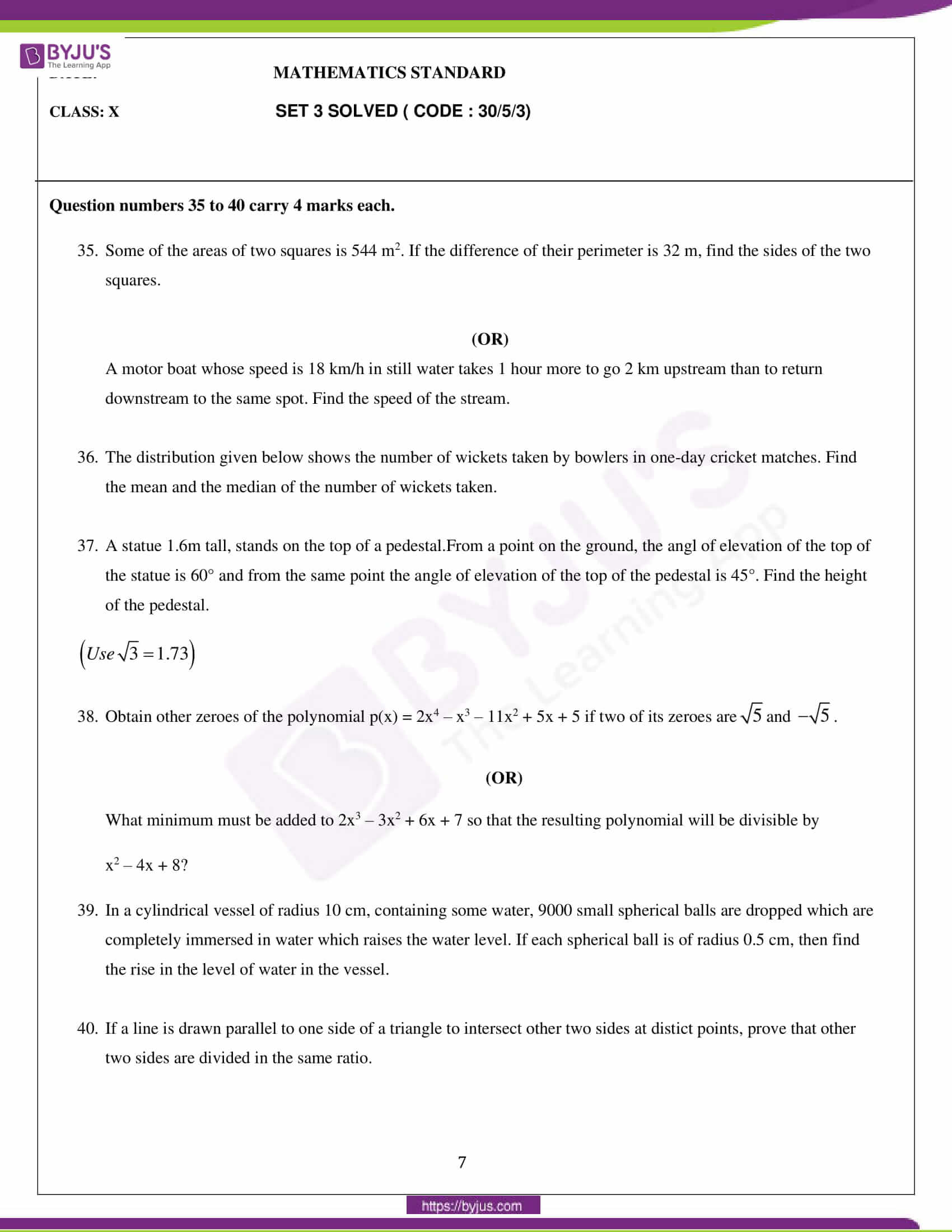### Maths Standard Set 3 (Code: 30/5/3) Question Paper 2020

General Instructions:

1. This question paper comprises four sections – A, B, C and D. This question paper carries 40 questions. All questions are compulsory.
2. Section A: Question numbers 1 to 20 comprises 20 questions of one mark each.
3. Section B: Question numbers 21 to 26 comprises 6 questions of two marks each.
4. Section C: Question numbers 27 to 34 comprises 8 questions of three marks each.
5. Section D: Question numbers 35 to 40 comprises 6 questions of four marks each.
6. There is no overall choice in the question paper. However, an internal choice has been provided in 2 questions of one mark, 2 questions of two marks, 3 questions of three marks and 3 questions of four marks. You have to attempt only one of the choices in such questions.
7. In addition to this, separate instructions are given with each section and question, wherever necessary.
8. Use of calculators is not permitted.

#### SECTION – A

Question numbers 1 to 20 carry 1 mark each.

Question numbers 1 to 10 are multiple choice questions.

Choose the correct option.

1. The value(s) of k for which the quadratic equation 2x2 + 5x + 2 = 0 has equal roots, is

(a) 4

(b) + 4

(c) – 4

(d) 0

2. Which of the following is not an A.P.?

(a) -1.2, 0.8, 2.8, ….

(b) $3, 3+\sqrt{2}, 3+2\sqrt{2}, 3+3\sqrt{2}$,..

(c) $\frac{4}{3}, \frac{7}{3}, \frac{9}{3}, \frac{12}{3}, ..$

(d) $\frac{-1}{5}, \frac{-2}{5}, \frac{-3}{5}, ..$

3. The radius of sphere (in cm) whose volume is 12π cm3, is

(a) 3

(b) $3\sqrt{3}$

(c) $3^{\frac{2}{3}}$

(d) $3^{\frac{1}{3}}$

4. The distance between the points (m, -n) and (-m, n) is

(a) $\sqrt{m^{2}+n^{2}}$

(b) m + n

(c) $2\sqrt{m^{2}+n^{2}}$

(d) $\sqrt{2m^{2}+2n^{2}}$

5. In Figure-1, from an external point P, two tangents PQ and PR are drawn to a circle of radius 4 cm with centre O. If $\angle QPR = 90^{0}$ , then length of PQ is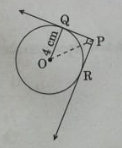(a) 3 cm

(b) 4 cm

(c) 2 cm

(d) $2\sqrt{2}$

6. On dividing a polynomial p(x) by x2 – 4, quotient and remainder are found to be x and 3 respectively. The polynomial p(x) is

(a) 3x2 + x – 12

(b) x3 – 4x + 3

(c) x2 + 3x – 4

(d) x3 – 4x – 3

7. In Figure-2, DE || BC. $\frac{AD}{DB} = \frac{3}{2}$ and AE = 2.7 cm, then EC is equal to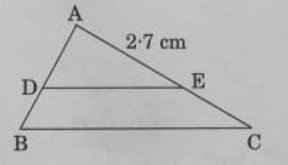(a) 2.0 cm

(b) 1.8 cm

(c) 4.0 cm

(d) 2.7 cm

8. The point on the x-axis which is equidistant from (-4, 0) and (10, 0) is

(a) (7, 0)

(b) (5, 0)

(c) (0, 0)

(d) (3, 0)

(OR)

The centre of a circle whose endpoints of a diameter are (-6, 3) and (6, 4) is

(a) (-8, -1)

(b) (4, 7)

(c) (0, 7/2)

(d) (4,7/2)

9. The pair of linear equations $\frac{3x}{2}+\frac{5y}{3}=7$ and 9x+10y=14 is

(a) consistent

(b) inconsistent

(c) consistent with one solution

(d) consistent with many solutions

10. In figure-3, PQ is tangent to the circle with center at O, at the point B.If $\angle AOB = 100^{0}, then\angle ABP$ is equal to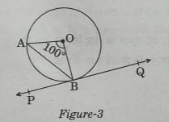(a) 50°

(b) 40°

(c) 60°

(d) 80°

Fill in the blanks in question number 11 to 15

11. Simplest form of $\frac{1 + tan^{2} A}{1 + cot^{2} A}$ is __________.

12. If the probability of an event E happening is 0.23, then $p(\bar{E})=$ ________.

13. All concentric circles are ___________ to each other.

14. The probability of an event that is sure to happen, is _______..

15. AOBC is a rectangle whose three vertices are A(0, – 3), O(0, 0) and B(4, 0). The length of its diagonals is ________________.

Answer the following question numbers 16 to 20.

16. Write the value of sin2 30° + cos2 60°.

17. Form a quadratic polynomial, the sum and product of whose zeroes are (-3) and 2 respectively.

(OR)

Can (x2 – 1) be a remainder while dividing x4 – 3x2 + 5x – 9 by (x2 + 3)?

18. Find the sum of the first 100 natural numbers.?

19. The LCM of two numbers is 182 and their HCF is 13. If one of the numbers is 26, find the other number.?

20. In figure – 4, the angle of elevation of the top of a tower from a point C on the ground, which is 30 m away from the foot of the tower, is 30°. Find the height of the tower.#### SECTION – B

Question numbers 21 to 26 carry 2 marks each.

21. A cone and a cylinder have the same radii but the height of the cone is 3 times that of the cylinder. Find the ratio of their volumes.

22. In figure – 6, a quadrilateral ABCD is drawn to circumscribe a circle. Prove that AB + CD = BC + AD.(OR)

In figure 7, find the perimeter of ΔABC, if AP = 12 cm.23. Find the mode of the following distribution:

 Marks: 0 – 10 10 – 20 20 – 30 30 – 40 40 – 50 50 – 60 Number of students: 4 6 7 12 5 6

24. In Figure-7, If PQ || BC and PR || CD, Prove that $\frac{QB}{AQ} = \frac{DR}{AR}$

25. Show that $5 + 2\sqrt{7}$ is an irrational number, where $\sqrt{7}$ is given to be an irrational number.

26. If A, B and C are interior angles of a ΔABC, then show that $cos\left ( \frac{B + C}{2} \right ) = sin\left ( \frac{A}{2} \right )$

#### SECTION – C

27. Prove that:

$\left ( sin^{4}\theta – cos^{4}\theta + 1 \right )cosec^{2}\theta = 2$

28. Find the sum: (-5)+(-8)+(-11)+…+(-230)

29. Construct a ΔABC with sides BC = 6 cm, AB = 5 cm and ∠ABC = 60°. Then construct a triangle whose sides are $\frac{3}{4}$ of the corresponding sides of ΔABC.

(OR)

Draw a circle of radius 3.5 cm. Take a point P outside the circle at a distance of 7 cm from the centre of the circle and construct a pair of tangents to the circle from that point.

30. In Figure-B, ABCD is a parallelogram, A semicircle with centre O and the diameter AB has been drawn and it passes through D. If AB = 12 cm and OD $\perp$ AB, then find the area of the shaded region. (use π = 3.14)

31. Read the following passage and answer the questions given at the end:

Diwali Fair

A game in a booth at a Diwali Fair involves using a spinner first. Then, if the spinner stops on an even number, the player is allowed to pick a marble from a bag. The spinner and the marbles in the bag are represented in Figure – 8. Prizes are given, when a black marble is picked. Shweta plays the same once.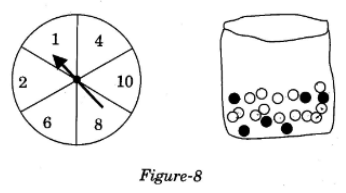(i) What is the probability that she will be allowed to pick a marble from the bag?

(ii) Suppose she is allowed to pick a marble from the bag, what is the probability of getting a prize, when it is given that the bag contains 20 balls out of which 6 are black?

32. A fraction becomes 1/3 when 1 is subtracted from the numerator and it becomes 1/4 when 8 is added to its denominator. Find the fraction.

(OR)

The present age of a father is three years more than three times the age of his son. Three years hence the father’s age will be 10 years more than twice the age of the son. Determine their present ages.

33. Find the ratio in which the y-axis divides the line segment joining the points (6, -4) and (-2, -7). Also find the point of intersection.

(OR)

Show that the points (7, 10), (-2, 5) and (3, -4) are vertices of an isosceles right triangle.

34. Use the Euelid Division Lemma to show that the square of any positive integer is either of the form 3q or 3q + 1 for some integer q.

#### SECTION – D

Question numbers 35 to 40 carry 4 marks each.

35. Some of the areas of the two squares are 544 m2. If the difference of their perimeter is 32 m, find the sides of the two squares.

(OR)

A motor boat whose speed is 18 km/h in still water takes 1 hour more to go 2 km upstream than to return downstream to the same spot. Find the speed of the stream.

36. The distribution given below shows the number of wickets taken by bowlers in one-day cricket matches. Find the mean and the median of the number of wickets taken.

37. A statue 1.6m tall, stands on the top of a pedestal.From a point on the ground, the angle of elevation of the top of the statue is 60° and from the same point the angle of elevation of the top of the pedestal is 45°. Find the height of the pedestal.( use $\sqrt{3}$ = 1.73 )

38. Obtain other zeroes of the polynomial p(x) = 2x4 – x3 – 11x2 + 5x + 5 if two of its zeroes are $\sqrt{5} and \sqrt{-5}$

(OR)

What minimum must be added to 2x3 – 3x2 + 6x + 7 so that the resulting polynomial will be divisible by x2 – 4x + 8?

39. In a cylindrical vessel of radius 10 cm, containing some water, 9000 small spherical balls are dropped which are completely immersed in water which raises the water level. If each spherical ball is of radius 0.5 cm, then find the rise in the level of water in the vessel.

40. If a line is drawn parallel to one side of a triangle to intersect the other two sides at distinct points, prove that the other two sides are divided in the same ratio.

CBSE Class 10 Mathematics Basic Question Paper 2020 – Coming Soon!

We hope this information on CBSE Class 10 Mathematics Question Papers 2020 helped students in their exam preparation. To get the CBSE Class 10 Previous Years Question papers with solutions, students can click here. Keep learning and Download BYJU’S App to access interactive Maths and Science videos.